## How to Statistic Pass 4 Out of 5 Rounds in a Competition by Excel Functions

Suppose you are counting the numbers of participants who are the winners in a competition. And the passing line is provided as pass 4 out of 5 rounds of games. You need to know if participant pass the game or not firstly. If you are familiar with the functions in Excel and can use them expertly, you can work out this issue easily. By the way, if you are confused of choosing and applying which function among all Excel functions, this article will help you to solve your problem.

In this article, through explaining the example below, we will introduce you to apply IF and COUNTIF functions together to return win or lose for each participant. You can get the basic knowledge and usage of these two functions in this article.

## EXAMPLE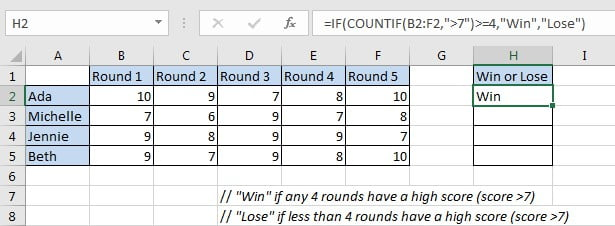In this case, we want to know if participants win or lose the game. “Win” is returned if participant passed four rounds of games with a high score; otherwise “Failed” is returned.

In this article, to approach our goal, we applied IF function with COUNTIF function inside.

## SOLUTION

In this instance, the final output in “Win of Lose” column is “Win” or “False”, the result depends on if participants passed any four rounds of games with high a high score. To output either A or B based on one condition, we can apply IF function here. This function is frequently used in the situations like ‘to return A or B by a given precondition”.

To count number of rounds with a high score, we can select COUNTIF function as it can count with one condition properly.

Besides, COUNTIF has some related functions like COUNT and COUNTIFS. COUNT function returns number of cells which contain a numeric value; COUNTIF is COUNT+IF, it returns number of cells with one condition or criterion; if there are multiple criteria, we can apply COUNTIFS.

## FORMULA with IF & COUNTIF FUNCTION

In H2, we input the formula =IF(COUNTIF(B2:F2,”>7″)>=4,”Win”,”Lose”). After typing, press Enter, verify that “Win” is displayed in H2. As Ada has a high score in round-1, round-2, round-4 and round-5, she passed the game with 4-rounds high scores, so “Win” is returned for her.Drag down handle to fill other cells with the same formula in “Win or Lose” column.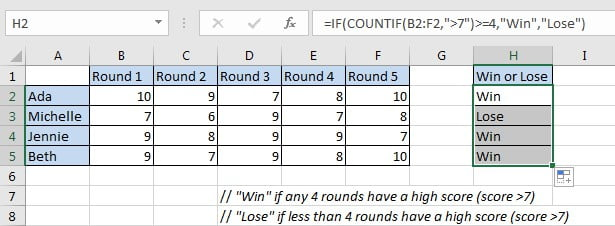Michelle lose the game as she only passes the round-3 and round-5 with a high score.

## FUNCTION INTRODUCTION

In this instance, returning “Win” or “False” depends on the result of whether participants passed four rounds of games with a high score, the final output “Win” of “False” comes from IF function. To determine if participants passed the game with 4 or more high scores, we applied COUNTIF function here.

a. COUNTIF returns the number of cells which contain a number and the number meets one condition or criterion. The condition or criterion is determined by our input in “criteria” argument.

Syntax:

`=COUNTIF(range, criteria)`

Example.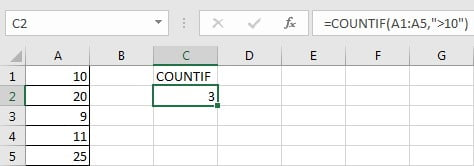b. IF function returns “true value” or “false value” based on the result of provided logical test. It is one of the most popular function in Excel.

Syntax:

`=IF(logical_test,[value_if_true],[value_if_false])`

Example.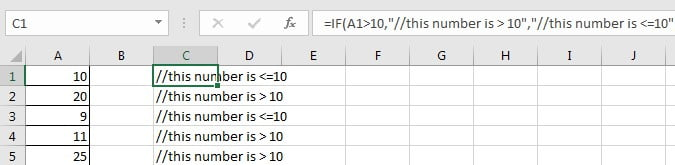## FORMULA EXPLANATION

`=IF(COUNTIF(B2:F2,">7")>=4,"Win","Lose")`

// logical test is “COUNTIF(B2:F2,”>7″)>=4”

// value of true is “Win”

// value of false is “Lose”

c. COUNTIF(B2:F2,”>7″) is used for counting the number of cells from range B2:F2 with the condition number in cell should be “greater than 7”. In this case, this expression is used for counting the rounds number with a high score 8, 9 or 10 for Ada. Expand values in B2:F2, we get below expression:

COUNTIF({10,9,7,8,10},”>7″) // returns 4

d. COUNTIF returned value “4” comes to the logical expression “COUNTIF(B2:F2,”>7″)>=4” inside IF function. In this logical test “4>=4” is true, so the value of true “Win” is returned by this formula finally.

=IF(4>=4,”Win”,”Lose”) // returns “Win”

## EXPAND

If we add two new conditions that round-1 and round-2 must pass with high score, current formula cannot satisfy our demand. Now, the passing line for “Win” is updated to must meet below three conditions:

1. The initial condition “COUNTIF(B2:F2,”>7″)>=4”.
2. Round-1 has a high score “B2>7”.
3. Round-2 has a high score “C2>7”.

Here, we can apply another function AND to concentrate the three conditions in one expression. AND function can return a “TRUE” if all conditions are met.

Update formula to =IF(AND(COUNTIFS(B2:F2,”>7″)>=4,B2>7,C2>7),”Win”,”Lose”).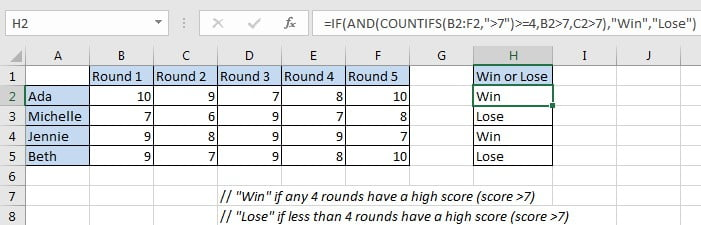### Related Functions

• Excel COUNTIF function
The Excel COUNTIF function will count the number of cells in a range that meet a given criteria. This function can be used to count the different kinds of cells with number, date, text values, blank, non-blanks, or containing specific characters.etc.= COUNTIF (range, criteria)…
• Excel COUNTIFS function
The Excel COUNTIFS function returns the count of cells in a range that meet one or more criteria. The syntax of the COUNTIFS function is as below:= COUNTIFS(criteria_range1, criteria1, [criteria_range2, criteria2]…)…
• Excel IF function
The Excel IF function perform a logical test to return one value if the condition is TRUE and return another value if the condition is FALSE. The IF function is a build-in function in Microsoft Excel and it is categorized as a Logical Function.The syntax of the IF function is as below:= IF (condition, [true_value], [false_value])….
• Excel AND function
The Excel AND function returns TRUE if all of arguments are TRUE, and it returns FALSE if any of arguments are FALSE.The syntax of the AND function is as below:= AND (condition1,[condition2],…)…

## How to Calculate Weekly Average by Formula in Excel

In our daily life we often create tables for recording the money we spend for lunch or dinner or something else per date. This can help us know the total cost or average cost per date or other unit clearly via some statistic related functions in excel, and then we can cut back on unnecessary expenses and save our money.

Normally, one method for statistic in excel is creating pivot table. we can create a pivot table to auto calculate the total cost or average cost base on the values recorded in original table, and we can do filter by date or month or quarter to show different average cost for different period. Details see below screenshot.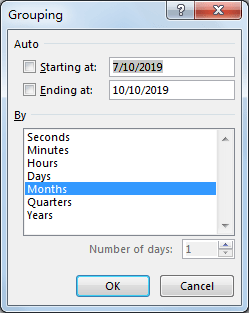Obviously, Week is not listed in default grouping date list.

If you want to know the method for counting average data by week (without using pivot table), you can read below article, it can help you to solve your question.

## Calculate Weekly Average by Formula in Excel

In Pre-Condition:

Prepare a simple table for demonstration. See screenshot below. You can see that we spend 10 or 5 or other values for lunch on different dates, some dates are included in one week. We want to count the weekly average for spend.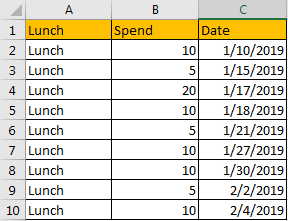Step 1: Create another table with three columns Week No., Year and Average. This table is used for recording the week number, year and the weekly average for launch spend.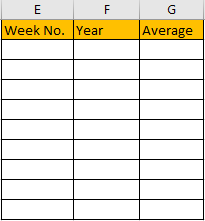Step 2: In E2, enter the formula =WEEKNUM(C2,2).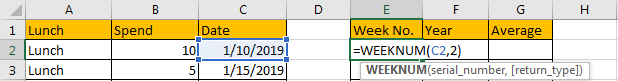For function WEEKNUM, we set the second parameter as 2, that means week begins on Monday.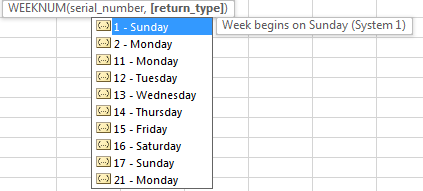Step 3: Click Enter to get returned value. We get 2 in this case. That means the date belongs to the second week in year 2019.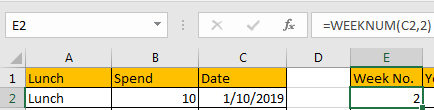Step 4: In F2, enter the formula =Year(C2). We can get Year 2019.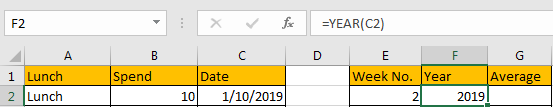Step 5: In G2, enter the formula =IF(AND(E2=E1,F2=F1),””,AVERAGEIFS(\$B\$2:\$B\$10,\$E\$2:\$E\$10,E2,\$F\$2:\$F\$10,F2)). Then we get the returned value 10.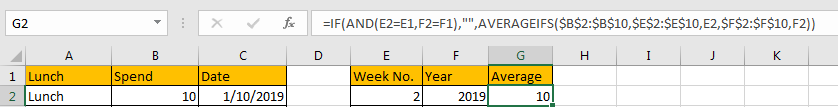Comment: In this formula, we use AVERAGEIFS function to calculate the average by specific criteria, and use IF function to classify dates by week and then get according average. If (E2=E1,F2=F1) is true, then we get blank value, otherwise we will get the weekly average.

Step 6: Drag the fill handle to fill the following cells in the table. We get weekly average values for all weeks.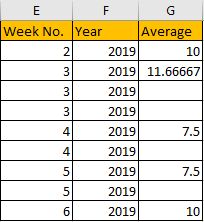Comment: If you want to ignore Year, you can just enter the formula =AVERAGEIFS(\$B\$2:\$B\$10,\$E\$2:\$E\$10,E2). Then we can get below table. You can depend on your requirement to determine whether add or remove criteria.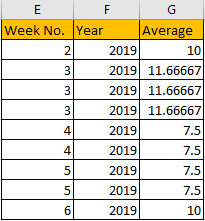### Related Functions

• Excel WEEKNUM function
The Excel WEEKNUM function returns the week number of a specific date, and the returned value is ranging from 1 to 53.The syntax of the WEEKNUM function is as below:=WEEKNUM (serial_number,[return_type])…
• Excel IF function
The Excel IF function perform a logical test to return one value if the condition is TRUE and return another value if the condition is FALSE. The IF function is a build-in function in Microsoft Excel and it is categorized as a Logical Function.The syntax of the IF function is as below:= IF (condition, [true_value], [false_value])….
• Excel AND function
The Excel AND function returns TRUE if all of arguments are TRUE, and it returns FALSE if any of arguments are FALSE.The syntax of the AND function is as below:= AND (condition1,[condition2],…)…

## How to Check If value Is between Two Numbers in Excel

This post will guide you how to check if a cell value is between two numbers in Excel. How do I build an IF statement that test if a cell value is between two given values in formula in Excel.

## Check Value If It Is between Two Numbers

Assuming that you have list of data in range A1:C4, which contain numbers. And you need to test if a numeric value in Cell C2 is between two numbers in Range: A2:B2. How to do it. You can use IF function and the AND function to build an IF statement to check values. Like this:

`=IF(AND(C2>A2,C2<B2),"True","False")`

Type this formula into a blank cell which you want to place the result, and press Enter key on your keyboard. Then drag the AutoFill Handle down to cell C4 to apply this formula.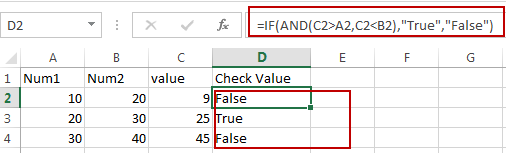Note: this formula is only valid when the Cell value in A2 is smaller than the cell value in B2.

You can also use another formula based on the AND function, the MIN function and the MAX function to achieve the same result. Like this:

`=AND(C2>MIN(A2,B2),C2<MAX(A2,B2))`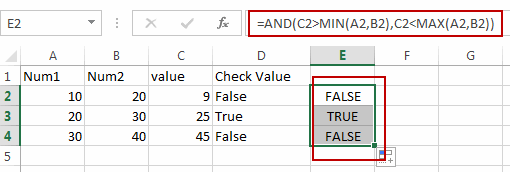### Related Functions

• Excel MIN function
The Excel MIN function returns the smallest numeric value from the numbers that you provided. Or returns the smallest value in the array.The MIN function is a build-in function in Microsoft Excel and it is categorized as a Statistical Function.The syntax of the MIN function is as below:= MIN(num1,[num2,…numn])….
• Excel MAX function
The Excel MAX function returns the largest numeric value from the numbers that you provided. Or returns the largest value in the array.= MAX(num1,[num2,…numn])…
• Excel IF function
The Excel IF function perform a logical test to return one value if the condition is TRUE and return another value if the condition is FALSE. The IF function is a build-in function in Microsoft Excel and it is categorized as a Logical Function.The syntax of the IF function is as below:= IF (condition, [true_value], [false_value])….
• Excel AND function
The Excel AND function returns TRUE if all of arguments are TRUE, and it returns FALSE if any of arguments are FALSE.The syntax of the AND function is as below:= AND (condition1,[condition2],…)…

## Ignoring Blank or Zero Cells with Conditional formatting

This post will guide you how to make conditional formatting ignore blank cells or zero cell in Excel. How do I force blank cells or zero cells to be ignored in conditional formatting in Excel. How to ignore blank cells or blank cells when applying conditional formatting in Excel.

## Ignoring Blank with Conditional Formatting

When you create conditional formatting rules for a list of selected data, and you want to ignore all Blank cells, How to achieve it. Just do the following steps:

#1 select the source data that you want to apply the conditional Formatting.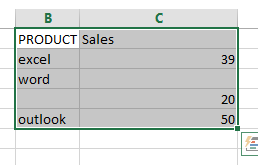#2 go to HOME tab, click Conditional Formatting command under Styles group. And select New Rule from the popup menu list. And the New Formatting Rule dialog will open.

#3 select Use a formula to determine which cells to format in the Select a Rule Type section.

#4 type the following formula into the Format values where this formula is true text box. Click Ok button.

=ISBLANK(B1)=TRUE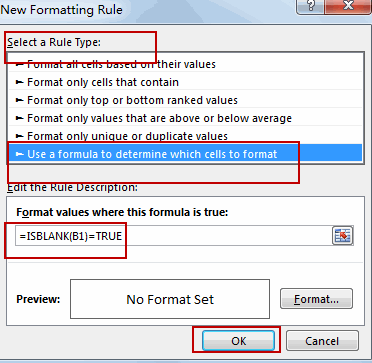Note: the B1 is the first cell of the selected range of cells.

## Ignoring zero with Conditional Formatting

If you want to ignore zero values with conditional Formatting, you can do the following steps:

#1 select the range of cells C2:C5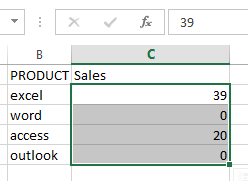#2 go to HOME tab, click Conditional Formatting command under Styles group, and select New Rule, and the New Formatting Rule dialog will open.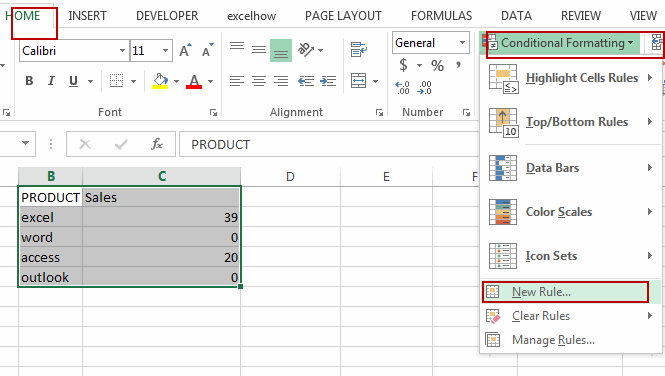#3 select Use a formula to determine which cells to format in the Select a Rule Type section.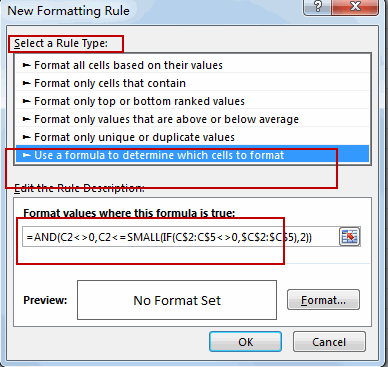#4 type the following formula into the Format values where this formula is true text box.

=AND(C2<>0,C2<=SMALL(IF(C\$2:C\$5<>0,\$C\$2:\$C\$5),2))

#5 click Format button, and switch to Fill tab in Format Cells dialog, select one color as the background color. Click Ok button.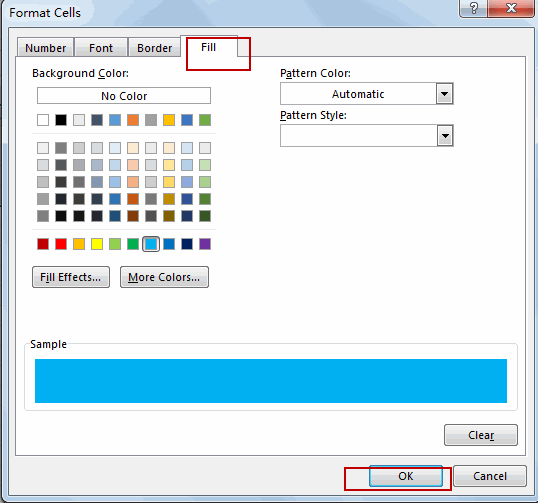#6 click Ok button. You will see that all zero values are ignored by conditional formatting.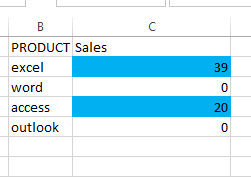### Related Functions

• Excel ISBLANK function
The Excel ISBLANK function returns TRUE if the value is blank or null.The syntax of the ISBLANK function is as below:= ISBLANK (value)…
• Excel IF function
The Excel IF function perform a logical test to return one value if the condition is TRUE and return another value if the condition is FALSE. The IF function is a build-in function in Microsoft Excel and it is categorized as a Logical Function.The syntax of the IF function is as below:= IF (condition, [true_value], [false_value])….
• Excel SMALL function
• The Excel SMALL function returns the smallest numeric value from the numbers that you provided. Or returns the smallest value in the array.The syntax of the SMALL function is as below:=SMALL(array,nth) …
• Excel AND function
• The Excel AND function returns TRUE if all of arguments are TRUE, and it returns FALSE if any of arguments are FALSE.The syntax of the AND function is as below:= AND (condition1,[condition2],…)…

## Returning Value if the Dates Fall between Two Dates

This post will guide you how to calculate value between two dates if the dates falls those two dates in Excel. How do return a value if the dates fall between two given dates in excel. How to use a formula to determine whether a date falls between two dates in Excel. How to check if a date falls between a range of two dates in Excel.

## Determine Whether a Date Falls between Two Dates

Assuming that you have a list of data that contain dates in one column or range, and you want to check those dates if fall between two given dates in range C1:D1. How to achieve it. You can create a formula based on the IF function, and the AND function. Like this:

=IF(AND(A1>\$C\$1,A1<\$D\$1),TRUE,FALSE)

Type this formula into Cell B1, and press Enter key in your keyboard, and then drag the AutoFill Handle from Cell B1 to B5.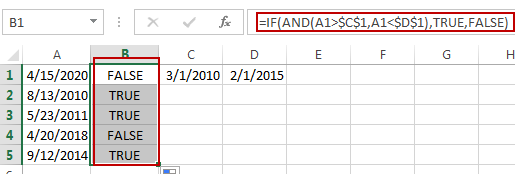If the data falls between the given two dates, and this formula return TRUE, otherwise, return FALSE.

### Related Functions

• Excel IF function
The Excel IF function perform a logical test to return one value if the condition is TRUE and return another value if the condition is FALSE. The IF function is a build-in function in Microsoft Excel and it is categorized as a Logical Function.The syntax of the IF function is as below:= IF (condition, [true_value], [false_value])….
• Excel AND function
The Excel AND function returns TRUE if all of arguments are TRUE, and it returns FALSE if any of arguments are FALSE.The syntax of the AND function is as below:= AND (condition1,[condition2],…) …

## Check If Multiple Cells are Equal In Excel

This post will guide you how to check if the values of multiple cells are equal with a formula in Excel. How do I verify that multiple cellsare the same in Excel. How to compare three or more cells in Excel to see if they are the same with a formula.

## Check If Multiple Cells are Equal

Assuming that you have a list of data in range A1:C1, and you want to compare if these cells are equal, if so, then return True, otherwise, return False. How to achieve it.
You need to create an Excel array formula based on the AND function and the EXACT function. Just like this:

`=AND(EXACT(A1:C1,A1))`

Type this formula into a blank cell, and press Ctrl +Shift +Enter shortcuts in your keyboard.

###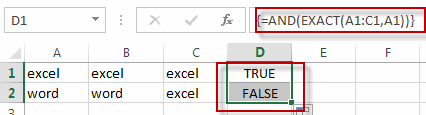There is another formula based on the COUNTIF function to achieve the same result. Like this:

`=COUNTIF(A1:C1,A1)=3`

### Related Functions

• Excel AND function
The Excel AND function returns TRUE if all of arguments are TRUE, and it returns FALSE if any of arguments are FALSE.The syntax of the AND function is as below:= AND (condition1,[condition2],…) …
• Excel COUNTIF function
The Excel COUNTIF function will count the number of cells in a range that meet a given criteria. This function can be used to count the different kinds of cells with number, date, text values, blank, non-blanks, or containing specific characters.etc.= COUNTIF (range, criteria)…
• Excel EXACT function
The Excel EXACT function compares if two text strings are the same and returns TRUE if they are the same, Or, it will return FALSE.The syntax of the EXACT function is as below:= EXACT (text1,text2)…

## Find Duplicate Values in Two Columns

This tutorial will show you how to find duplicate values in two given columns in Excel. Or How to compare two columns and find duplicate entries in Microsoft Excel worksheet. And you will learn two methods to compare columns so that the duplicate values can be highlighted or listed in a range of Cells.

Assuming that you need to compare two columns (Column A and Column B) to get the duplicate values in your worksheet, you can find duplicate values in two columns with Excel Formula, or Excel VBA Macro code.

## Method 1: Find duplicate values in two columns with Excel Formula

To compare two given columns and find duplicate values in Excel, you can use a combination of the IF function, the ISERROR function, and the MATCH function to create an Excel formula. So you can use the following formula:

`=IF(ISERROR(MATCH(A1,\$B\$1:\$B\$4,0))," ",A1)`

Now you need to type this formula in Cell C1, press Enter key, drag AutoFill Handle down to Cell C2:C4, you will see all of the duplicated values are displayed in Column C.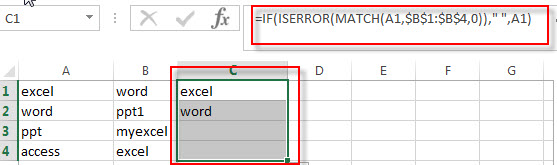## Method 2: Find duplicate values in two columns with VBA Macro code

If you are familiar with the programming language and you can use a Visual Basic Macro to compare the value in two columns and then find duplicate values, just refer to the following steps:

1# click on “Visual Basic” command under DEVELOPER Tab.2# then the “Visual Basic Editor” window will appear.

3# click “Insert” ->”Module” to create a new module4# paste the below VBA code into the code window. Then clicking “Save” button.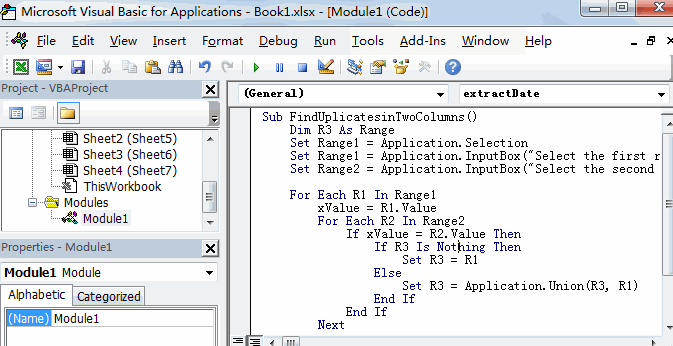```Sub FindUplicatesinTwoColumns()
Set Range1 = Application.Selection
Set Range1 = Application.InputBox("Select the first range in one column:", "FindUplicatesinTwoColumns", Range1.Address, Type:=8)
Set Range2 = Application.InputBox("Select the second range in another column:", "FindUplicatesinTwoColumns", Type:=8)

For Each R1 In Range1
xValue = R1.Value
For Each R2 In Range2
If xValue = R2.Value Then
If R3 Is Nothing Then
Set R3 = R1
Else
Set R3 = Application.Union(R3, R1)
End If
End If
Next
Next
R3.Interior.ColorIndex=3

End Sub```

5# back to the current worksheet, then run the above excel macro.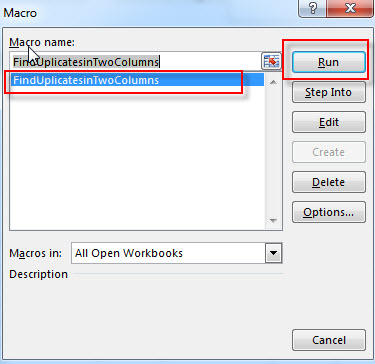6# Select the first range A1:A4 in Column A, click OK button, then select the second range B1:B4 in Column B, click OK button.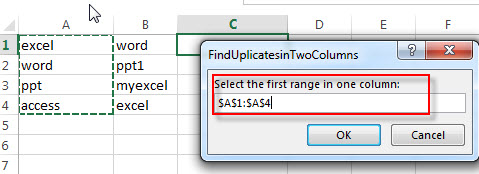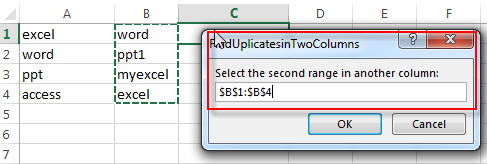7# let’s see the result: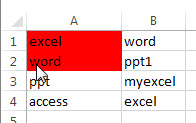## Method 3: Find duplicate values in two columns with Conditional Formatting feature

You can use conditional formatting with on a formula based on the COUNTIF function and the AND function to find the duplicate values in two specified columns and then highlighted them. Just do it following:

1# Select the entire Column A via click on the Column Header and then the column A will be highlighted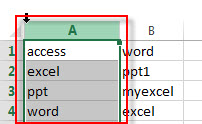2# on the HOME tab, click the Conditional Formatting command under Styles group. Then select New Rules… from the drop-down menu list.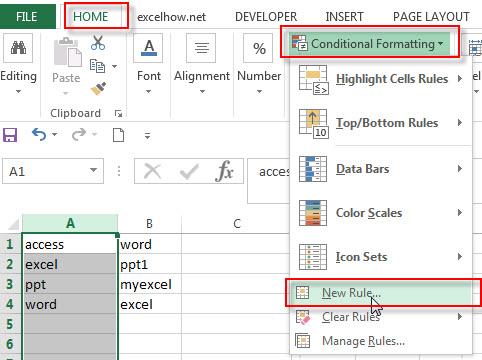3# select Use a formula to determine which cells to format as Rule Type in the New Formatting Rule window

4# Type the following formula in the Format values where this formula is true: box

`=COUNTIf(\$B:\$B, \$A1)`

5# click the Format… button, then the Format Cells window will appear.

6# in the “Format Cells” window, switch to the Fill tab, choose the background color, and then click OK button.

7#you will be back to the New Formatting Rule windows and you can check a preview of the formatting you have selected. Then click OK button.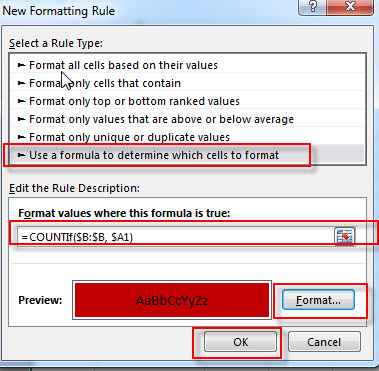Then the conditional formatting rule will be applied to all values in two columns and highlighted the duplicate values.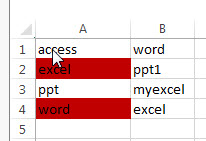### Related Functions

• Excel IF function
The Excel IF function perform a logical test to return one value if the condition is TRUE and return another value if the condition is FALSE. The IF function is a build-in function in Microsoft Excel and it is categorized as a Logical Function.The syntax of the IF function is as below:= IF (condition, [true_value], [false_value])….
• Excel ISERROR function
The Excel ISERROR function used to check for any error type that excel generates and it returns TRUE for any error type, and the ISERR function also can be checked for error values except #N/A error, it returns TRUE while the error is #N/A. The syntax of the ISERROR function is as below:= ISERROR (value)….
• Excel MATCH function
The Excel MATCH function search a value in an array and returns the position of that item.The syntax of the MATCH function is as below:= MATCH  (lookup_value, lookup_array, [match_type])….
• Excel COUNTIF function
The Excel COUNTIF function will count the number of cells in a range that meet a given criteria. This function can be used to count the different kinds of cells with number, date, text values, blank, non-blanks, or containing specific characters.etc.= COUNTIF (range, criteria)…
• Excel AND function
The Excel AND function returns TRUE if all of arguments are TRUE, and it returns FALSE if any of arguments are FALSE.The syntax of the AND function is as below:= AND (condition1,[condition2],…)…

### Related Posts

• Highlight Rows
You will learn that how to change the color of the entire rows if the value of cells in a specified column meets your conditions, such as, if the value of cells is equal to or greater than a certain number or text values, then excel should be highlight entire rows or change a row color as you need.…
• Find Duplicate Rows
If you want to check the entire row that duplicated or not, if True, then returns “duplicates” value, otherwise, returns “no duplicates”. You can create a formula based on the IF function and the SUMPRODUCT function..…
• Highlight Duplicate Rows
this post will talk that how to highlight entire rows that are duplicates in excel 2016, 2013 or lower version. Or how to change the background color of duplicate rows..…
• Highlight duplicate values
this post will teach you how to highlight duplicate values in the range of cells in excel. Normally, you may be need to identify duplicate values with a range of cells in Excel. And there is one of the fasted way that is using conditional formatting feature in Microsoft Excel……

## Check if Cell Contains Certain Values but do not Contain Others Values

In the previous post, we only talked that how to check a cell if contains one of several values from a range in excel. And this post explains that how to check a cell if it contains certain values or contains one of several values but do not contain other certain values in another range or a list in excel.

## Check if Cell Contains Certain Values but do not Contain Others Values

Supposing that you have a list of text strings in the range B1:B3 and you need to check each Cell that if it contains one of several values in a range D2:D4 but do not contains any of values in range E2:E3.  If TRUE, then returns TRUE value, otherwise, returns FALSE value.

To check a cell to see if it contains certain string but do not contain others. You can use a combination of the AND function, the COUNT function and the SEARCH function to create a new array formula as follows:

`=AND(COUNT(SEARCH(\$D\$2:\$D\$4,B1))>0, COUNT(SEARCH(\$E\$2:\$E\$3,B1))=0 )`

Let’s see how this formula works:

= SEARCH(\$D\$2:\$D\$4,B1)

The SEACH function returns position of the first character of find_text in a text string. And this formula will search each value in the range D2:D4 inside within_text in Cell B1, then returns position of each text string in Cell B1, so it will return an array result like this:

`{1;7;12}`

= SEARCH(\$E\$2:\$E\$3,B1)

This SEARCH formula will search each value in range E2:E3 inside within_text in Cell B1, and then returns position of the first position of each find_text in Cell B1. When no match is found, the SEARCH function will return the #VALUE error. So it will return an array result like this:

`{#VALUE!;#VALUE!;1}`

=COUNT(SEARCH(\$D\$2:\$D\$4,B1))>0

The COUNT function will count the number of cells that contain numbers in an array returned by the SEARCH function. If it is greater than 0, then it indicated that at least one values is found in Cell B1.

=COUNT(SEARCH(\$E\$2:\$E\$4,B1))=0

If the number of cells that contain numbers is equal to 0, then it means that none of values can be found in Cell B1.

=AND(COUNT(SEARCH(\$D\$2:\$D\$4,B1))>0, COUNT(SEARCH(\$E\$2:\$E\$4,B1))=0 )

If both two COUNT function return TRUE value, the AND function returns TRUE. And if either COUNT function is FALSE, this formula returns FALSE.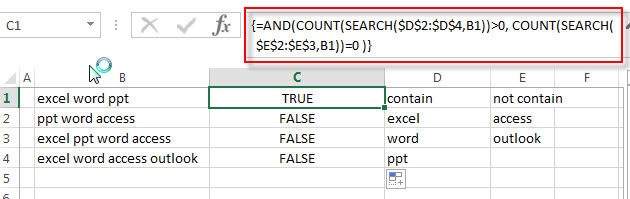### Related Functions

• Excel SEARCH function
The Excel SEARCH function returns the number of the starting location of a substring in a text string.The syntax of the SEARCH function is as below:= SEARCH  (find_text, within_text,[start_num])…
• Excel COUNT function
The Excel COUNT function counts the number of cells that contain numbers, and counts numbers within the list of arguments. It returns a numeric value that indicate the number of cells that contain numbers in a range…
• Excel AND function
The Excel AND function returns TRUE if all of arguments are TRUE, and it returns FALSE if any of arguments are FALSE.The syntax of the AND function is as below:= AND (condition1,[condition2],…)…

## Excel IF Function With Numbers

Normally, If you want to write an IF formula for numbers in combining with the  logical operators in excel, such as: greater than, less than, greater than or equal to, less than or equal to, equal to, not equal to.etc.

The generic IF formula with logical operator for number values is as below:

`=IF(B1>10,”good”,”bad”)`

## Excel IF formula for range of numbers

If you want to check if a cell values is between two values or checking for the range of numbers or multiple values in cells, at this time, we need to use AND or OR logical function in combination with the logical operator and IF function.

For example, you need to check if the value in cell B1 is between values in Cell A1 and A3, then we can write down the following excel IF formula:

`=IF(AND(B1>A1,B1<A3),"good","bad")`

We can enter the above formula into the formula bar in the cell C1 and then press the Enter key. And rag the Fill Handle to the range C1:C3.it will apply for the other cells for this formula.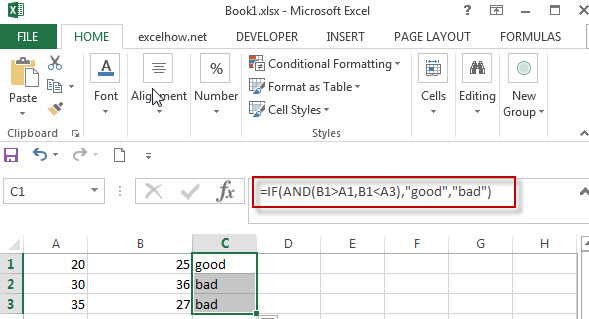## Excel IF formula for negative numbers

If you want to create an IF formula to display some text string in cells if the value in cell falls between the range negative numbers -10 to positive numbers 10.

Actually, whatever negative numbers or positive numbers, we still can use AND or OR logical function to create a logical test in IF formula. we can write down the following IF formula:

`=IF(AND(B1>-10, B1<10),”good”,”bad”)`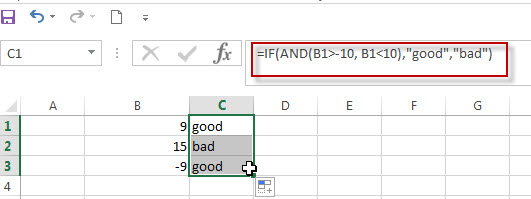Also, we can use another excel function ABS() to achieve the same results. Let’s see the below IF formula in combination with ABS function.

`=IF(ABS(B1)<10,”good”,”bad”)`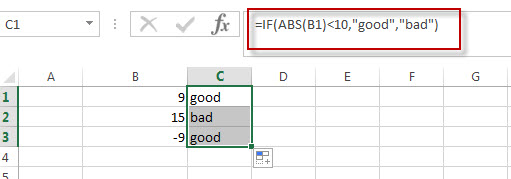### Related Formulas

• Excel IF formula with operator : greater than,less than
Now we can use the above logical test with greater than operator. Let’s see the below generic if formula with greater than operator:=IF(A1>10,”excelhow.net”,”google”) …
• Excel IF function with text values
If you want to write an IF formula for text values in combining with the below two logical operators in excel, such as: “equal to” or “not equal to”.
• Excel IF function with Dates
If you have a list of dates and then want to compare to these dates with a specified date to check if those dates is greater than or less than that specified date. …
• Excel IF Function With Numbers
If you want to check if a cell values is between two values or checking for the range of numbers or multiple values in cells, at this time, we need to use AND or OR logical function in combination with the logical operator and IF function…

### Related Functions

• Excel AND function
The Excel AND function returns TRUE if all of arguments are TRUE, and it returns FALSE if any of arguments are FALSE.The syntax of the AND function is as below:= AND (condition1,[condition2],…) …
• Excel IF function
The Excel IF function perform a logical test to return one value if the condition is TRUE and return another value if the condition is FALSE….
• Excel nested if function
The nested IF function is formed by multiple if statements within one Excel if function. This excel nested if statement makes it possible for a single formula to take multiple actions…
• Excel ABS function
The Excel ABS function returns the absolute value of a number

## Excel IF formula with NOT logical function

This post will guide you how to use excel if formula with NOT logical function in Microsoft excel.

## NOT Logical Function

The syntax of NOT logical function is as follow:

`=NOT(logical_value)`

The NOT logical function will return the reversed logical value. If the logical_value is TRUE, then FALSE is returned. If the logical_value is FALSE, then TRUE is returned. Let’s see the below two examples:

```=NOT(FALSE)
=NOT(2+1=3)```

Both of the above excel formulas will return TRUE.

## Excel IF Formula Combining With NOT

If you want to check several test conditions in an excel formula,then take different actions. You can use NOT function in combination with the AND or OR logical function in excel IF function.

Let’s see the following excel if formula:

`=IF(NOT(OR(B1="red",B1="black")),TRUE,FALSE)`

In the above formula, the excel formula will exclude both red and blank colors. It will check cells in column B if the values is NOT red or blank. TRUE or FALSE will be returned.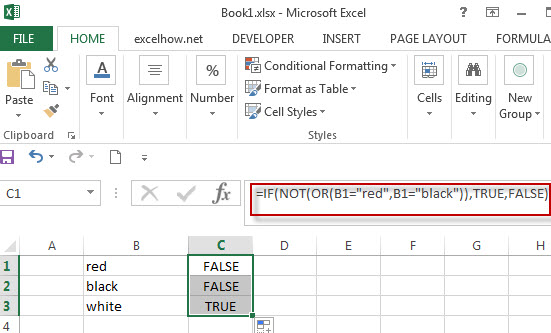Actually, we also can use the Not equal to (<>) logical operator to achieve the same requirement , For example, we can write down the following excel IF formula to reflect the above logic test.

`=IF(AND(B1<>”red”,B1<>”black”)),TRUE,FALSE)`

## NOT Function combining with ISBLANK function

As you know, the function ISBLANK(B1) will return TRUE if the cell B1 is blank. If we use NOT function to enclose ISBLANK(B1) function, it will reverse the result, if the cell is blank, then returns FALSE.

Let’s create a nested IF statement with the NOT and ISBLANK functions as follow:

`=IF(NOT(ISBLANK(B1)),B1*5,””)`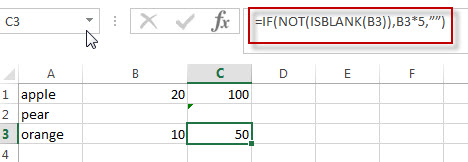For above IF statement, IF the cell B1 is not blank, then multiply the number in B1 by 5.we can enter the above formula into cell C1, and then drag the Fill Handle to the range C1:C3.it will apply for the other cells for this formula.

### Related Functions

• Excel OR function
The Excel OR function returns TRUE if any of the conditions are TRUE in logic test. Otherwise, it returns FALSE.
• Excel AND function
The Excel AND function returns TRUE if all of arguments are TRUE, and it returns FALSE if any of arguments are FALSE.The syntax of the AND function is as below:= AND (condition1,[condition2],…) …
• Excel IF function
The Excel IF function perform a logical test to return one value if the condition is TRUE and return another value if the condition is FALSE….
• Excel nested if function
The nested IF function is formed by multiple if statements within one Excel if function. This excel nested if statement makes it possible for a single formula to take multiple actions…
• Excel ISBLANK function
The Excel ISBLANK function returns TRUE if the value is blank or null.The syntax of the ISBLANK function is as below:= ISBLANK (value)…
• Excel NOT function
The Excel NOT function returns the opposite of a given logical or Boolean value. For example, if you supplied with the value TRUE, the NOT function will return FALSE; If you supplied with the value FALSE, and the NOT function will TRUE. The syntax of the NOT function is as below:=NOT(logical)…

## Excel IF formula with operator : greater than,less than

This post will guide you how to use if function with “greater than”,  “greater than or equal to”, ”less than” and “less than or equal to” logical operators in excel.

## Greater than, Greater than or equal to, Less than, less than or equal to

The “greater than”(>)operator will compare the size of two different values in cells and then return TRUE if the first value in cells compared is larger than the second values in cells. FALSE if they are not.

The “greater than or equal to” (>=) operator will return TRUE if the first value in cells is larger than the second or if the two values are equal.

The “Less than” operator returns TRUE if the first value in cell is smaller than the second value in cells.

The “Less than or equal to” operator returns TRUE if the first value in cell is smaller than the second of the two values are equal.

Those excel comparison operators mostly used to compare numbers, date and time values.

Also, we can use those operators to compare text string, and it will compare the value of the first letters in the text string. And if the first letters are same, it will compare the second letters and so on.

Let’s see the below excel if formula with greater than operator:

 Formula example Description =A1>10 Returns True if a number value in cell A1 is greater than 10, FALSE if they are not. =A1>=(B1*2) Returns True if a number value in cell A1 is greater than or equal to the value of  B1*2, FALSE if they are not. =A1

## The Generic IF formula with Greater than operator

Now we can use the above logical test with greater than operator. Let’s see the below generic if formula with greater than operator:

`=IF(A1>10,”excelhow.net”,”google”)`

If you want to take the different action when a value in cells is greater than a certain value (it can be number, text, date or another function), then you can use the IF function with greater than operator to make a logical test and return one value if the logical test condition is true, or return another value if the logical test condition is false.

For the above IF formula, it just tests the value in cell A1 to check if the value is greater than 10. If TRUE, the IF formula will return “excelhow.net”. If FALSE, the IF function will return “google”.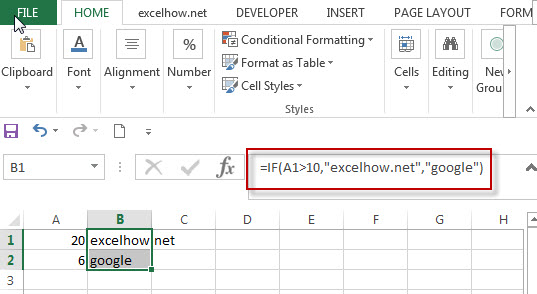You might want to return “excelhow.net” if the test condition is TRUE. Or return nothing if the test returns FALSE. You can use an empty string (“”) as False value in the IF function, see below if formula:

`=IF(A1>10,”excelhow.net”,””)`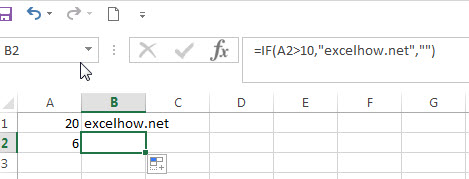## The Generic IF formula with Greater than or equal to operator

`=IF(A1>=(B1*2),”excelhow.net”,”google”)`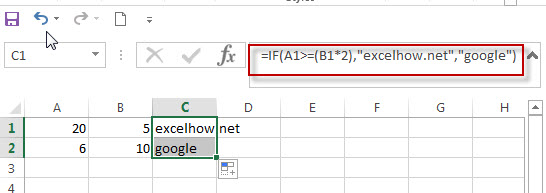## The Generic IF formula with less than operator

`=IF(A1<DATEVALUE(“10/30/2018”),”excelhow.net”,”google”)`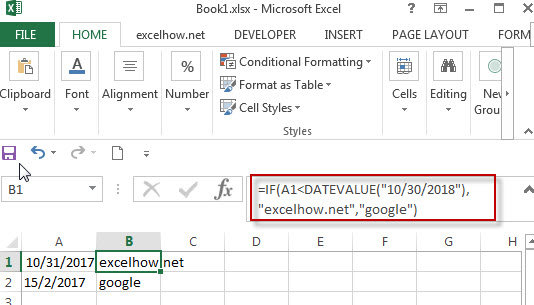## The Generic IF formula with Greater than operator

`=IF(A1<=”excelhow”,”excelhow.net”,”google”)`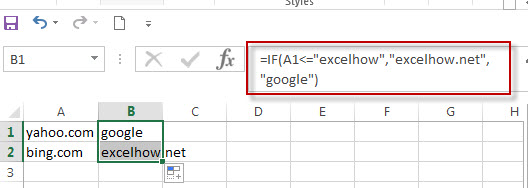### Related Functions

• Excel AND function
The Excel AND function returns TRUE if all of arguments are TRUE, and it returns FALSE if any of arguments are FALSE.The syntax of the AND function is as below:= AND (condition1,[condition2],…) …
• Excel IF function
The Excel IF function perform a logical test to return one value if the condition is TRUE and return another value if the condition is FALSE….
• Excel nested if function
The nested IF function is formed by multiple if statements within one Excel if function. This excel nested if statement makes it possible for a single formula to take multiple actions…
• Excel DATEVALUE Function
The Excel DATEVALUE function returns the serial number of date. And it can be used to convert a date represented as text format into a serial number that recognizes as a date format.The syntax of the DATEVALUE function is as below:=DATEVALUE(date_text)…

## Excel IF formula with AND & OR logical functions

If you want to test the result of cells based on several sets of multiple test conditions, you can use the IF function with the AND and OR functions at a time.

Assuming that you have the following test logic to check the exam scores:

Logic test1: 60<=B1<=70

Logic test2: 60<=C1<=70

If any of the above conditions are met, then mark the result as “good”, otherwise, mark the result as “bad”. We need to use two AND functions to reflect the above two logical test and enclose them in the OR function as one logical test in the Excel IF formula. Let’s write down the below IF formula with AND & OR logical functions:

`=IF(OR(AND(B1>=60,B1<=70),AND(C1>=60,C1<=70)),"good","bad") `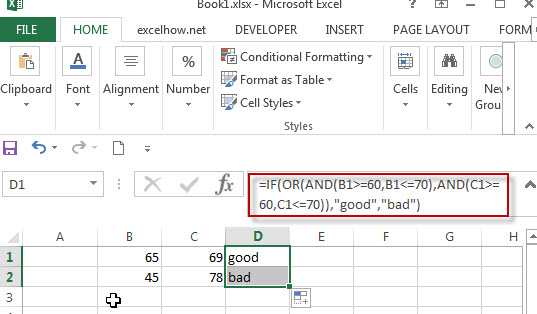Let’s see another IF formula with AND and OR functions as follows:

`=IF(AND(B1="tom",OR(C1>=60,D1>=60)),"pass","")`

When B1 is equal to “tom”, and either C1 is greater than or equal to “60” or D1 is greater than or equal to 60, the formula returns “pass”. Otherwise, the formula will return an empty string.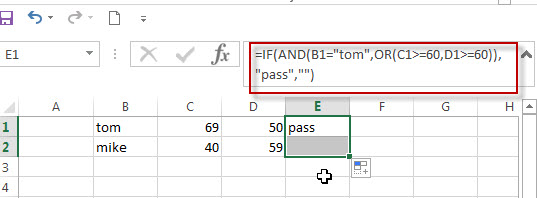If we just want to check the cells where the value in column B is “tom” AND the score in column C is greater than or equal to 60. If you want to return TRUE, you can use the below logical test statement with the AND function:

`AND(B1="tom",C1>=60)`

Next, we can extend the above statement, we want to check the cells if the values in column B is “tom” AND the scores in column C or column D is greater than or equal to 60. Then we can nest the OR function in the above AND function like below:

`AND(B1="tom",OR(C1>=60,D1>=60))`

### Related Formulas

• Excel IF formula with equal to logical operator
The “Equal to” logical operator can be used to compare the below data types, such as: text string, numbers, dates, Booleans.  This section will guide you how to use “equal to” logical operator in excel IF formula with text string value and dates value…
• Excel IF formula with OR logical function
If you want to check if one of several conditions is met in your excel workbook, if the test is TRUE, then you can take certain action. You can use the IF function combining with OR function to construct a condition statement…
• Excel IF formula with AND logical function
You can use the IF function combining with AND function to construct a condition statement. If the test is FALSE, then take another action…

### Related Functions

• Excel AND function
The Excel AND function returns TRUE if all of arguments are TRUE, and it returns FALSE if any of arguments are FALSE.The syntax of the AND function is as below:= AND (condition1,[condition2],…) …
• Excel OR function
The Excel OR function used to test multiple conditions and returns TRUE if any of the conditions are TRUE. Otherwise, it will return FALSE. The syntax of the OR function is as below:=OR(logical1, [logical2], …)…
• Excel IF function
The Excel IF function perform a logical test to return one value if the condition is TRUE and return another value if the condition is FALSE….
• Excel nested if function
The nested IF function is formed by multiple if statements within one Excel if function. This excel nested if statement makes it possible for a single formula to take multiple actions…

## Excel IF formula with OR logical function

This post will guide you how to use IF function combining with OR logical function in Excel.

If you want to check if one of several conditions is met in your excel workbook, if the test is TRUE, then you can take certain action. You can use the IF function combining with OR function to construct a condition statement. If the test is FALSE, then take another action.

## Excel formula using IF & OR function

The syntax of OR function in excel is as follow:

`=OR(condition1,[condition2],...)`

The Excel OR function returns TRUE if any of the conditions are TRUE in logic test. Otherwise, it returns FALSE.

Now we want to check the results of two exam scores where the first score in cell A1 is greater than or equal to 40 OR the second score in cell B1 is greater than or equal to 50. If any of conditions are TRUE, then returns the text of “good”, and if FALSE returns “bad”.

Based on the above logic, we can write down the below IF formula:

`=IF(OR(A1=”40”,B1=”50”),”good”, ”bad”)`

We can enter the above formula into cell D1, and then drag the Fill Handle to the range D1:D3.it will apply for the other cells for this formula.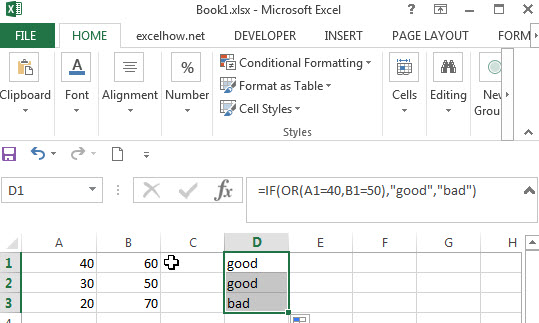So the difference from the IF&AND formula discussed in the previous post is that the IF&OR formula will return TRUE if any of conditions are true.

In the above formula, we just want to check both A1 and B1 cells, the Excel will take an action when the result of test condition is TRUE. In this case, we will add “good” text in the D1 cell. If the test condition is FALSE, assuming that you do not want to display anything in the cell D1, then we can write down the following excel if formula:

`=IF(OR(A1=”40”,B1=”50”),”good”,””)`

Normally, you can use as many AND/OR logical functions as your logic requires, but there is limitation for argument numbers, we can follow the below limitation for excel formula:

1. No more than 255 arguments in Microsoft excel 2016,2013,2010 and 2007
2. No more than 8192 characters in Microsoft excel 2016,2013,2010 and 2007
3. No more than 30 arguments and the length of formula should not exceed 1024 characters in Microsoft excel 2003 or lower version.

### Related Functions

• Excel OR function
The Excel OR function returns TRUE if any of the conditions are TRUE in logic test. Otherwise, it returns FALSE.
• Excel AND function
The Excel AND function returns TRUE if all of arguments are TRUE, and it returns FALSE if any of arguments are FALSE.The syntax of the AND function is as below:= AND (condition1,[condition2],…) …
• Excel IF function
The Excel IF function perform a logical test to return one value if the condition is TRUE and return another value if the condition is FALSE….
• Excel nested if function
The nested IF function is formed by multiple if statements within one Excel if function. This excel nested if statement makes it possible for a single formula to take multiple actions…

## Excel IF formula with AND logical function

This post will guide you how to use IF function combining with AND logical function in Excel.

If you want to check if a value in cell match two or more conditions at the same time, if the test is TRUE, then you can take certain action. You can use the IF function combining with AND function to construct a condition statement. If the test is FALSE, then take another action.

## Excel formula using IF & AND function

The syntax of AND function in excel is as follow:

`=AND(condition1,[condition2],...)`

The Excel AND function returns TRUE if all of arguments are TRUE, and it returns FALSE if any of arguments are FALSE.

Now we want to check the results of two exam scores where the first score in cell A1 is greater than or equal to 60 AND the second score in cell B1 is greater than or equal to 80. If both conditions are TRUE, then returns the text of “good”, and if FALSE returns “bad”. Based on the above logic, we can write down the below IF formula:

`=IF(AND(A1=60,B1=80),"good","bad")`

We can enter the above formula into cell D1, and then drag the Fill Handle to the range D1:D3.it will apply for the other cells for this formula.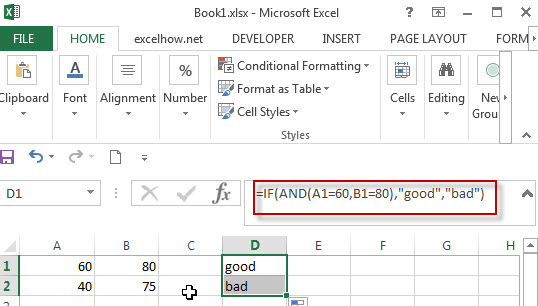The logic test will check the two different cells in the above IF formula, if we can run two or more tests on the same cell. Of course yes, for example, if you want to check cell A1 if the value in cell A1 is greater than 60 but less than or equal to 70. If the test is TRUE, then take one action.

The logical test we can use for above logic as below:

`=AND(A1>60, A1<=70)`

IF formula:

`=IF(AND(A1>60,A1<=70),”good”,””)`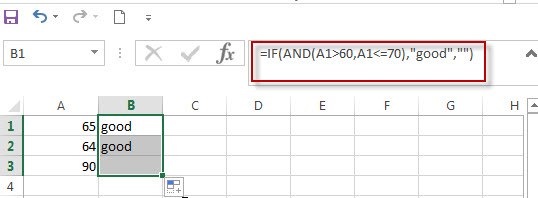In this formula, if the test is FALSE, we just add an empty string (“”), IF the test is TRUE, the test string of “good” will appear.

Note: The AND function will check all test conditions in excel, even if one of the already tested conditions has a value of FALSE. This behavior is different with other programming languages. If any previous test conditions return FALSE, the subsequent conditions will not be tested.

For example: If the value of Cell B1 is 0, then the below IF&AND formula will return error message as “Divide by Zero Error”.

`=IF(AND(B1<>0,(1/B1)>0.2),”A”,”D”))`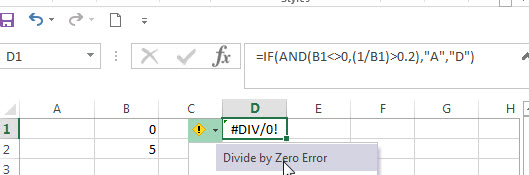As mentioned above, the AND function will check all conditions. To avoid this issue, we can use a nested IF function and check if the value of Cell B1 is not equal to 0 in the first IF statement.

The nested if formula is as below:

`=IF(B1<>0,IF((1/B1>0.2),”A”,”D”))`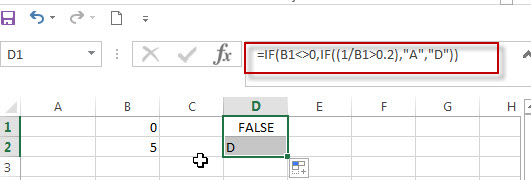### Related Formulas

• Excel IF formula with equal to logical operator
The “Equal to” logical operator can be used to compare the below data types, such as: text string, numbers, dates, Booleans.  This section will guide you how to use “equal to” logical operator in excel IF formula with text string value and dates value…
• Excel IF formula with OR logical function
If you want to check if one of several conditions is met in your excel workbook, if the test is TRUE, then you can take certain action. You can use the IF function combining with OR function to construct a condition statement…
• Excel IF formula with AND & OR logical functions
If you want to test the result of cells based on several sets of multiple test conditions, you can use the IF function with the AND and OR functions at a time…

### Related Functions

• Excel AND function
The Excel AND function returns TRUE if all of arguments are TRUE, and it returns FALSE if any of arguments are FALSE.The syntax of the AND function is as below:= AND (condition1,[condition2],…) …
• Excel IF function
The Excel IF function perform a logical test to return one value if the condition is TRUE and return another value if the condition is FALSE….
• Excel nested if function
The nested IF function is formed by multiple if statements within one Excel if function. This excel nested if statement makes it possible for a single formula to take multiple actions…

## Excel nested if statements with ranges

Many people usually asked that how to write an excel nested if statements based on multiple ranges of cells to return different values in a cell? How to nested if statement using date ranges? How to use nested if statement between different values in excel 2013 or 2016?

This post will guide you how to understand excel nested if statements through some classic examples.

## Nested IF statements based on multiple ranges

Assuming that you want to reflect the below request through nested if statements:

a) If 1<B1<0, Then return 0.1

b) If 0.99<B1<5, then return 0.15

c) If 4.99<B1<15, then return 0.2

d) If 14.99<B1<30, then return 0.5

So if B1 cell was “14.5”, then the formula should be returned “0.2” in the cell.

From above logic request, we can get that it need 4 if statements in the excel formula, and there are multiple ranges so that we can combine with logical function AND in the nested if statements.  The below is the nested if statements that I have tested.

`=IF(AND(B1>0,B1<1),0.1,IF(AND(B1>0.99, B1<5),0.15, IF(AND(B1>4.99,B1<15),0.2, IF(AND(B1>14.99,B1<30),0.5,””))))`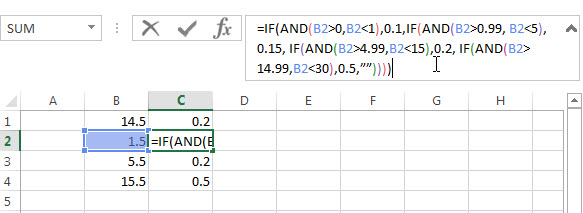If you don’t want to use AND function in the above nested if statement, can try the below formula:

`=IF(B1<1,0.1, If(B1<5,0.15,IF(B1<15,0.2,IF(B1<30,0.5,””))))`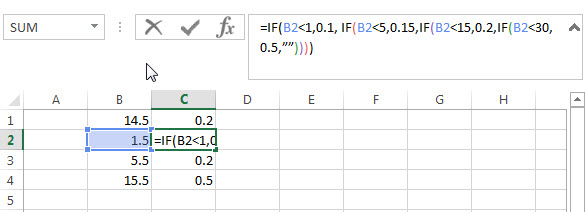## Nested IF Statement Using Date Ranges

I want to write a nested IF statement to calculated the right Quarter based one the criteria in the below table.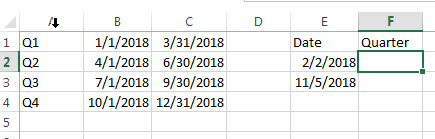According to the request, we need to compare date ranges, such as: if B1<E2<C1, then return A1.

So we can consider to use AND logical function in the nested if statement. The formula is as follows:

`=IF(AND(E2>B1,E2<C1),A1,IF(AND(E2>B2,E2<C2),A2,IF(AND(E2>B3,E2<C3),A3,IF(AND(E2>B4,E2<C3),A4))))`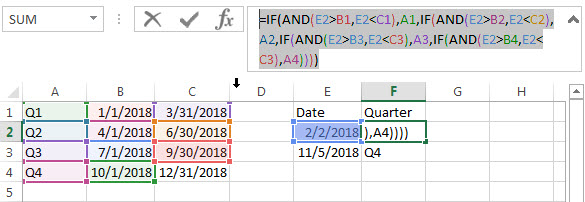Or we can use INDEX function and combine with MATCH Function to get the right quarter.

`=INDEX(\$A\$1:\$A\$4,MATCH(E2,\$B\$1:\$B\$4,1))`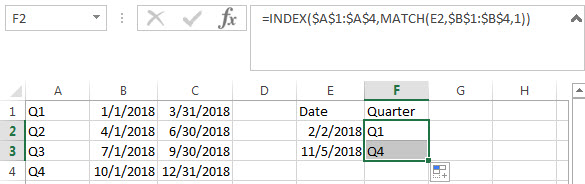## Nested IF Statements For A Range Of Cells

If you have the following requirement and need to write a nested IF statement in excel:

If any of the cells A1 to C1 contain “excelhow”, then return “excelhow” in the cell E1.

If any of the cells A1 to C1 contain “google”, then return “excelhow” in the cell E1.

If any of the cells A1 to C1 contain “ibm”, then return “ibm” in the cell E1.

If any of the cells A1 to C1 contain “Cloud”, then return “ibm” in the cell E1.

How to check if cell ranges A1:C1 contain another string, using “COUNTIF” function is a good choice.

Countif function: Counts the number of cells within a range that meet the given criteria

Let’s try to test the below nested if statement:

`=IF(OR(COUNTIF(A3:C3,"excelhow")>0,COUNTIF(A3:C3,"google")>0),"excelhow",IF(OR(COUNTIF(A3:C3,"ibm")>0,COUNTIF(A3:D3,"cloud")>0),"ibm",""))`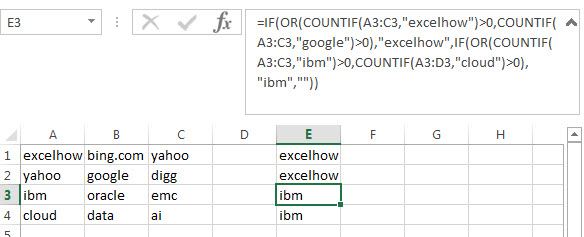## Nested IF Statement between different values

Assuming you have the following different range values, if the B1 has the value 65, then expected to return “under average”in cell C1, if the Cell B1 has the value 75, then return “average” in cell C1. And if the Cell B1 has the value 85, then return “above average” in the cell C1.

 0-70 under average 71-80 average 81-100 above average

How do I format the nested if statement in Cell C1 to display the right value? Just try to use the below nested if function or using INDEX function.

`=IF(B1< 0,"",IF(B1<= 70, "under average",IF(B1<=80, "Average", IF(B1<=100,"Above Average",""))))`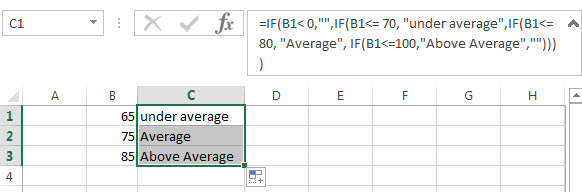### Related Functions

• Excel IF function
The Excel IF function perform a logical test to return one value if the condition is TRUE and return another value if the condition is FALSE. The IF function is a build-in function in Microsoft Excel and it is categorized as a Logical Function.The syntax of the IF function is as below:= IF (condition, [true_value], [false_value])….
• Excel nested if function
The nested IF function is formed by multiple if statements within one Excel if function. This excel nested if statement makes it possible for a single formula to take multiple actions…
• Excel AND function
The Excel AND function returns TRUE if all of arguments are TRUE, and it returns FALSE if any of arguments are FALSE.The syntax of the AND function is as below:= AND (condition1,[condition2],…) …
• Excel COUNTIF function
The Excel COUNTIF function will count the number of cells in a range that meet a given criteria.This function can be used to count the different kinds of cells with number, date, text values, blank, non-blanks, or containing specific characters.etc.The syntax of the COUNTIF function is as below:= COUNTIF (range, criteria) …
• Excel INDEX function
The Excel INDEX function returns a value from a table based on the index (row number and column number)The INDEX function is a build-in function in Microsoft Excel and it is categorized as a Lookup and Reference Function.The syntax of the INDEX function is as below:= INDEX (array, row_num,[column_num])…
• Excel MATCH  function
The Excel MATCH function search a value in an array and returns the position of that item.The MATCH function is a build-in function in Microsoft Excel and it is categorized as a Lookup and Reference Function.The syntax of the MATCH function is as below:= MATCH  (lookup_value, lookup_array, [match_type])….

## Excel Nexted IF Functions (Statements) Tutorial (15 IF Formulas examples)

This tutorial will guide you how to use nested Excel IF function  (include multiple If statements in excel formula) with syntax and provide about 15 nested IF formula examples with the detailed explanation in Microsoft excel.

## Description

The Excel IF function perform a logical test to return one value if the condition statement is TRUE and return another value if the condition statement is FALSE. The IF function is a build-in function in Microsoft Excel and it is categorized as a Logical Function.

The excel if function just only test one condition and if you want to deal with more than one condition and return different actions depending on the result of the tests,  then you need to include several IF statements (functions) in one excel IF formula, these multiple IF statements are also called Excel Nested IF formula(Nested IFs). It’s also similar with IF-THEN-ELSE statement.

The nested IF function is formed by multiple if statements within one Excel if function. This excel nested if statement makes it possible for a single formula to take multiple actions.

The most recent versions of Excel (Excel 2016, Excel 2013, Excel 2010 and Excel 2007) allow 64 IF functions (statements) in one formula, and it was only possible up to 7 nested IF functions (statements) in Excel 2003 and lower version.

## Syntax

The syntax of Nested IF function is as below:

`=IF(Condition_1,Value_if_True_1,IF(Condition_2,Value_if_True_2,Value_if_False_2))`

Where the Nested IF function argument is:

Condition_1 –  The condition that you want to test  in the first IF statement.

Value_if_True_1 – The value that is returned if first IF statement is True. If the condition_1 return False, then move into the next IF function.

Condition_2 – The condition that you want to test in the second IF statement.

Value_if_True_2 – The value that is returned if second IF statement is True.

Value_if_False_2 – The value is returned if second IF statement is False.

This is equivalent to the following IF THEN ELSE statement:

```IF Condition_1 THEN
Value_if_True_1
ELSEIF Condition_2
Value_if_True_2
ELSE
Value_if_False_2
END IF```

## Examples of Nested IF function (Statement) in Excel

The below examples will show you how to use Excel Nested IF function with the detailed explanation of their syntax and logic.

Example 1#  The most basic Nested IF function with one level of nesting

If you want to write a nested if function to test the following calculation logic for assigning value in the cell A1.

```IF A1 =="excelhow" THEN
return "excel"
ELSEIF A1 == "excelhow.net" THEN
return "MS excel"
ELSE
return "MS"
END IF```

we can write a nested IF function based on the above logic as follows:

`=IF(A1="excelhow", "excel", IF(A1="excelhow.net","MS excel","MS"))`

In above Nested IF formula, the nested if function is shown with bold style and it is inside the outer IF function. we can see that if A1 is not equal to the “excelhow“, then the second nested  IF function will be test. and if second IF condition statement return FALSE, then the entire IF function will return “MS” value.

Example 2#  The Nested IF function with two levels of nesting

Assuming that you want to test more than one condition statement in the above nested if function, add one condition to test if the value of the cell A1 reference is equal to “www.excelhow.net” , If TRUE, then return “Microsot excel“.

The calculation Logic is as below:

```IF A1 =="excelhow" THEN
return "excel"
ELSEIF A1 == "excelhow.com" THEN
return "MS excel"
ELSEIF A1 == "www.excelhow.net" THEN
return "Microsoft excel"
ELSE
return "MS"
END IF```

we can add one more IF statement inside the second IF function in the above excel nested if formula in example1. let’s see the below nested if function with tow level nesting:

`=IF(A1="excelhow", "excel", IF(A1="excelhow.com","MS excel",IF(A1="www.excelhow.net","Microsoft excel","MS")))`

In the above nested excel IF formula, the first nested if function is marked with red color, and the second nested excel if function is marked with blue color.

If the both first and second conditions are False and the third IF condition will be check, IF A1 is equal to “www.excelhow.net” , then return “Microsoft excel“, or the entire nested IF formula will return “MS“.

Example 3#  Describes the each IF function contained in the nested IF function

​We will use one typical example of excel nested if function to describe each IF function included in the nested if function.

Assuming that you need to assign a grade based on a score with the following test conditions:

Let’s write a nested if function based on the above logic as follows:

`=IF(A1>=80, "excellent", IF(A1>=60, "good", IF(A1>0, "bad", "no valid score")))`

For the above excel if formula, lets describe it for each IF function statement.

1# IF Cell A1 is greater than or equal to 80, then the formula will return “excellent” or move to the second If function.

2# If Cell A1 is greater than or equal to 60, then the formula will return  “good” or move to the third IF function

3# IF Cell A1 is greater than 0, then the formula will return “bad”, or the IF function will return “no valid score”.

Example 4#  Describes each If function in the excel Nested IF Statement (another simple example of if function)

Let’s describe the below Nested IF Function example:

`=IF(A1<=6,60, IF(A1<=8,80,IF(A1<=10,100,200)))`

a) If cell A1 is equal to 6 or less than 6, then return value 60 in cell C1. Let’s see below screenshot.

b) If Cell A1 is greater than 6 and less or equal to 8, then retrun value 80 in Cell C1.

c) If cell A1 is greater than 8 and less than or equal to 10, then return value 100 in cell C1.

d) If cell A1 is greater than 10 , then the Nested if function will return the last value “200”in cell C1.

Example 5#  Excel Nested IF function with arithmetic operator (+, -, * , /)

Assuming that you want to write a Nested If function to reflect the following logic tasks:

a) IF Cell A1 is less than 10, then multiply by 10.

b) IF Cell A1 is greater than or equal to 10 but less than 20, then add 20

c) IF Cell A1 is greater than or equal to 20 but less than 30, then minus 20

d) IF Cell A1 is greater than or equal to 30 but less than 50, then divided by 20

The nested IF formula is as follows:

`=IF(A1<10,A1*10,IF(A1<20,A1+20,IF(A1<30,A1-20,IF(A1<50,A1/20))))`

a1) if Cell A1 is less 10 (A1=5), then the first If condition matched and will take multiply action, A1 * 10=5*10=50, so it will return 50 in the cell C1

b1) if Cell 10<=A1<20 (A1=15), then the second if condition matched and will take add action, A1+20=15+20=35, so it will return 35 in the cell C1.

c1) if Cell 20<=A1<30(A1=25), then the third if condition matched and will take minus action, A1-20=25-20=5, so it will return 5 in the cell C1.

d1) if Cell 30<=A1<50 (A1=35), then the forth if condition matched and will take divide action, A1/20=35/20=1.75, so it will return 1.75 in the cell C1.

Example 6#  Excel Nested IF function with logical function –AND

Assuming that you need a nested if function to reflect the following logic:

a) IF A1+B1 is less than 10, then return 10

b) IF A1+B1 is greater than 10 but less than or equal to 20, then return 20

c) IF A1+B1 is greater than 20 but less than or equal to 30, then return 30.

d) IF A1+B1 is greater than 30, then return 200.

Let’s write the following nested IF formula in the cell C1:

`=IF(A1+B1<10,10, IF(A1+B1<=20,20, IF(A1+B1<=30,30,200)))`

The above formula just use basic nested IF function syntax, we also can use logic function to re-write it, the nested if formula with AND function is as follows:

`=IF((A1+B1)<10,10,IF(AND((A1+B1)>10,(A1+B1)<=20),20, IF(AND((A1+B1)>20,(A1+B1)<=30),30,200)))`

The above nested IF formula combined with two AND function.

In the second IF Statement, AND((A1+B1)>10,(A1+B1)<=20) will check if 10<A1+B1<=20, If TRUE, then the formula will return 20.

In the third IF Statement, AND((A1+B1)>20,(A1+B1)<=30) will check if 20<A1+B1<=30, If TRUE, then the formula will return 30.

Example 7# Excel Nested IF function with logical function –OR

Assuming that you need a nested if function to reflect the following logic:

a) IF Cell A1=5, return A1/B1

b) IF Cell A1=10, return A2/B2

c) IF Cell A1=15 or A1=20, return A3/B3

In Cell C1, we can write the below nested if formula based on the above conditions.

`=IF(A1=5,A1/B1,IF(A1=10,A2/B2,IF(OR(A1=15,A1=20),A3/B3)))`

One OR function be used in the above excel nested if function, it will check if A1=15 or A1=20, if TRUE, then return A3/B3.

Example 8#  Excel nested if function with text and logical function AND

Wrote a nested if function with text to reflect the following logic:

a) If Cell A1=”E” and Cell B1=5, then return “Excel”

b) If Cell A1=”P” and Cell B1=3, then return “PPT”

c) If Cell A1=”W” and Cell B1=4, then return “Word”

d) Else return “Access”

In Cell C1, try to enter into the following excel nested If formula with AND function:

`=IF(AND(A1="E",B1=5),"Excel", IF(AND(A1="P",B1=3),"PPT", IF(AND(A1="W",B1=4),"Word","Access")))`

Example 9#  Excel nested if function with ISBLANK function and logical function AND

a) If you want to wrote a nested if function with ISBLANK function and logical function AND to reflect the following logic:

b) If both Cell A1 and Cell B1 are empty, then return “”

c) If only Cell A1 is empty, then return B1-today()

d) If both two cells A1 and B1 are not empty, then return “excel” string.

In Cell C1, use the following excel nested If formula with ISBLANK and AND function:

`=IF(AND(ISBLANK(A1),ISBLANK(B1)),"",IF(ISBLANK(A1),B1-TODAY(),"excel"))`

Example 10#  Using nested IF functions to check grade level based on student’s score(multiple IF statements)

The logic is as below:

We will write a nested If function that reflect the above logic, and will check if the score is below 50, If TRUE, it is considered as “Fail”. If FALSE, move into the next IF statement to test if the score is between 51 and 60 and it is considered as “Grade C”. If False, we will move into another IF statement to check if the score is between 61 and 80, IF True and it is considered as “Grade B”. If FASLSE, just check the rest conditions.

We can use a nested if formula as follows:

`=IF(B2<=50,"Fail",IF(AND(B2>=51, B2<=60), "Grade C", IF(AND(B2>=61, B2<=80),"Grade B",IF(AND(B2>=81,B2<=95),"Grade A", "Grade A+"))))`

Example 11#  Nested IF function for checking two Empty Cells

Let’s see the below image a product table of a company (need to create an excel table firstly):

a) If we need to check both “Price” cell and “Quantity” cell are empty, If True, then return empty. If the only “price” cell is empty only, IF True, return empty.

b) If the only “Quantity” cell is empty, IF True, return empty.

c) If both “price” and “Quantity” are not empty, then return multiply Price * Quantity as subtotal value.

So To check both “Price” and Quantity cells, we can use table header name as condition variable to test each Price cells or Quantity cells, so we can write the nested if formula as follows:

`=IF([Price]="","",IF([Quantity]="","",[Price]*[Quantity]))`

Just using the above excel if formula in the subtotal cells, the formula will check the first IF statement if Price Cell is empty, IF TRUE, then will return empty (“”) in the subtotal cell. IF FALSE, then move to the next IF statement and so on. Last, IF neither cell is empty, then will return the value of multiply [Price]*[Quantity] in subtotal cell.

Of course, we can use another nested if function to achieve the above calculation logic (easy to understand).

`=IF(ISBLANK(B2),"",IF(ISBLANK(C2),"", B2*C2))`

OR

`=IF(B2="","",IF(C2="","", B2*C2))`

## Nested IF Functions Order

There is one important thing that need us keep in mind when write Excel Nested IF Function, it is the order of nested IF function. It can nested up to 64 If statements, and how to order multiple IF condition statements, it is key point. Or the wrong result will be returned. The point is that excel nested if function will test the first if condition in the order, once any condition is met, and the subsequent if conditions will not be checked.

So let’s remember the below rules while writing excel nested if function:

• The most important condition First or Harder Test First

Let’s see the below example what it means:

Example 12#

There are two test conditions in the following excel nested if function:

`=IF(B1>=90,"excellent",IF(B1>=60,"good","bad"))`

When using this formula in the cell B3, If the amount in cell B1 is 95, then “excellent” would be returned. because it is greater than 90. And the second IF condition will not be evaluated.

However, if the order of nested if statment are reversed as follows:

`=IF(B1>=60,"good",IF(B1>=90,"excellent","bad"))`

The above formula would check for the condition B1>=60 first, if the amount in cell B1 is 95,  then the value “good” would be returned in cell B3. Because the Cell B1 match the first test condition, and it will not check the second if condition and will return the incorrect result.

## Nested IF Function Alternatives

To make your excel formulas more efficiency and fast, you can try to use the following alternatives to excel nested if function.

1)
Excel nested if function can be easily replaced with the VLOOUP, Lookup, INDEX/MATCH or CHOOSE functions.

Example 13#  Use VLookup function instead of nested IF function

Nested IF function:

`=IF(D1=39,"excelhow.net", IF(D1=45,"two",IF(D1=34,"google.com","")))`

Vlookup function:

`=VLOOKUP(39,A1:B3,2,FALSE)`

Example 14#  Use CHOOSE function instead of nested if function

Nested If unction:

`=IF(B1>=60,"good","bad")`

Using CHOOSE function as follows:

`=CHOOSE(IF(B1<60,1,2),"bad","good")`

2) Use IFS instead of nested if function

3) Use the CONCATENATE function or the concatenate operator (&).

Example 15#

Nested IF function:

`=IF(B1="A","excellent",IF(B1="B","good","bad"))`

Using CONCATENATE function as follows:

`=CONCATENATE(IF(B1=”A”,”excellent”,””),IF(B1=”B”,”good”,””),IF(B1=”C”,”bad”))`

Question1: Is there any tool to help write Excel formulas and nested Ifs?

This is an Excel formula with nested IF statements:

`=IF((B2="East"),4,IF((B2="West"),3,IF((B2="North"),2,IF((B2="South"),1,""))))`

To essentially accomplish this:

```If cell B2 = "East"
return "4"
ElseIf cell B2 = "West"
return "3"
ElseIf cell B2 = "North"
return "2"
ElseIf cell B2 = "South"
return "1"
Else
return ""```

Can Excel formulas be written in such a “more readable” manner and converted to the official syntax? Is there any tool to help write Excel formulas?

Answer: Excel Formula Formatter add-in by Rob van Gelder, mentioned at Daily Dose of Excel.

Excel’s formula bar ignores line feeds and white space, so you can Alt+Enter and spacebar to format the formulas however you like. I’ve tried it and I quickly stopped doing it. Too much spacebar-ing, especially if you need to edit.

Question2: I am working on an excel file, and i am trying to use a nested if formula to achieve what i would like.

i have two columns:A B.  And the condition is this: if the value in a2=a3, then check if the minus of b2 and b3 is certain value, and if it is, put a yes, else put a no. this will iterate till the end of the excel file.

so far here is what i have. not sure how to use the excel formulas. any help is much appreciated.

`if(a2=a3,b2-b3=5 or b2-b3=-5 or b2-b3=20 or b2-b3=-20, "yes", "no")`

Answer: you should be able to use the OR function within your nested if formula to test for “B2-B3=5,B2-B3=-5,B2-B3=20,B2-B3=-20” as follows:

`=IF(A2=A3,IF(OR(B2-B3=5,B2-B3=-5,B2-B3=20,B2-B3=-20),"yes","no"),"no")`

### Related Functions

• Excel IF function
The Excel IF function perform a logical test to return one value if the condition is TRUE and return another value if the condition is FALSE….
• Excel And function
The Excel AND function returns TRUE if all of arguments are TRUE, and it returns FALSE if any of arguments are FALSE…
• Excel Concat Function
The excel CONCAT function combines 2 or more strings or ranges together. The syntax of the CONCAT function is as below: =CONCAT (text1,[text2],…) …
• Excel Vlookup Function
The Excel VLOOKUP function lookup a value in the first column of the table and return the value in the same row based on index_num position.The syntax of the VLOOKUP function is as below:= VLOOKUP (lookup_value, table_array, column_index_num,[range_lookup])…
• Excel Choose Function
The Excel CHOOSE function returns a value from a list of values. The CHOOSE function is a build-in function in Microsoft Excel and it is categorized as a Lookup and Reference Function.The syntax of the CHOOSE function is as below:=CHOOSE (index_num, value1,[value2],…)…
• Excel ISBLANK function
The Excel ISBLANK function returns TRUE if the value is blank or null.The syntax of the ISBLANK function is as below:= ISBLANK (value)…

## Excel IF Function

This post will guide you how to use Excel IF function with syntax and examples in Microsoft excel.

## Description

The Excel IF function perform a logical test to return one value if the condition is TRUE and return another value if the condition is FALSE.

The IF function is a build-in function in Microsoft Excel and it is categorized as a Logical Function.

The IF function is available in Excel 2016, Excel 2013, Excel 2010, Excel 2007, Excel 2003, Excel XP, Excel 2000, Excel 2011 for Mac.

## Syntax

The syntax of the IF function is as below:

`= IF (condition, [true_value], [false_value])`

Where the IF function arguments are:
Condition -This is a required argument.  A user-defined condition that is to be tested.
True_value – This is an optional argument.  The value that is returned if condition evaluates to TRUE.
False_value – This is an optional argument.  The value that is returned if condition evaluates to FALSE.

The below examples will show you how to use Excel IF Function to return one value if the condition is TRUE or FALSE.

`=IF(B1>90, good, bad)`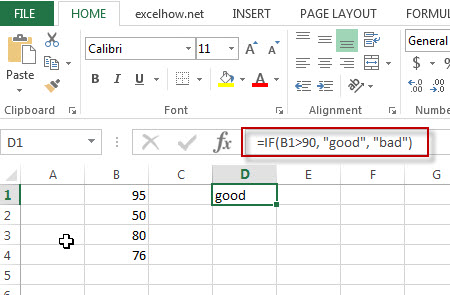Note: the above excel formula will test a condition “b1>90”, if the condition is true, then “good” will return or “bad” will return.

## Nested IF statements

The excel if function just only test one condition and if you want to deal with more than one condition and return different actions depending on the result of the tests,  then you need to include several IF statements (functions) in one excel IF formula, these multiple IF statements are also called Excel Nested IF formula(Nested IFs).

For example:

`=IF(A1>=80, "excellent", IF(A1>=60, "good", IF(A1>0, "bad", "no valid score")))`

More reading: Excel Nexted IF Functions (Statements) Tutorial

## Excel Logical operators

When you are writing an If statement, you may need to use any of the following excel logical operators:

 Operator Meaning Example Description = Equal To A1=B1 Returns True if a value in cell A1 is equal to the values in cell B1; FALSE if they are not. > Greater Than A1>B1 Returns True if a value in cell A1 is greater than the values in cell B1; FALSE if they are not. >= Greater Than or Equal to A1>=B1 Returns True if a value in cell A1 is greater than or equal to the values in cell B1; FALSE if they are not. < Less Than A1 Not Equal to A1<>B1 Returns True if a value in cell A1 is not equal to the values in cell B1; FALSE if they are not.

Let’s see some examples for logical operators in Excel formula:

 A1 B1 8 5

```
=(A1>B1)
Output: TRUE

=(A1<B1)
Output: FALSE

=(A1>=B1)
Output: TRUE

=(A1<>8)
Output: FALSE

=(A1<>B1)
Output: TRUE

=(IF A1>3, “TRUE”, “FALSE”)
Output: TRUE```

Question1: I want to write an IF Formula based on the below test criteria in the Microsoft excel.

If the number in Cell B1 is greater than 30, then I want it to return A

If the number in cell B1 is between 20 and 30, then I want it to return B

If the number in Cell B1 is below 20, then I want it to return C

The below formula is what I currently write:

`=IF(B1>30,"A",IF(B1<30,B1>20,"B","C"))`

When I entered the above formula in the Cell C1, and it will return an Error.

Answer:  You can use IF function in combination with AND function to reflect the above logic. So you can do it using the following formula:

`=IF(B1>30,"A",IF(AND(B1>=20,B1<=30),"B","C"))`

Or you can use another IF formula without AND function as follows:

`=IF(B1>30,"A",IF(B1<20,"C","B"))`

Question 2: I want to write a excel Formula for sales rep, If the sales are less than 10K, then the member will get no commission. If the sales are between 10 and 50K, then the member will get a 3% commission. If the sales are more than 50k, then the member will get 5% commission. Could you help me?

Answer: Based on the above description, we can use the following excel IF formula:

`=IF(B1<10,0,IF(B1<50,B1*3%,B1*5%))`

Question 3: I want to select students for the scholarship in school, and based on the student’s scores and attendance value, if the student scores more than 85 and has the attendance of more than 85%, then he/she will get the scholarship. Please help me how to write this formula based on my test criteria.

Answer: you can use the IF function with the AND function to check whether both of two conditions are met or not. If the test are met, then return “Yes”, otherwise returns “No”.

So you can use the following IF formula in Excel:

`=IF(AND(B1>85, C1>85%),"Yes","No")`

Question 4:  I am trying to write an excel formula based on the following logic:

I want to enter a formula in Cell C1 that will Add B1, B2 and B3 or multiply B1 by 1, multiply B2 by 2, multiply B2 by 3, which action will be taken based on what you put in Cell D1.

Appreciated for any help. Thanks

Answer: Based on the above logic description, you need to use SUM function to count the sum of B1, B2 and B3. We can use the following IF formula:

`=IF(D1="Add”,SUM(B1,B2,B3),IF(D1="Multiply",B1*B2*B3,"ERROR"))`

Question 5:  I am trying to write a formula in excel to check the employee number field and returns the relevant band of employee. I already have one IF formula, but I always return the first value of “A”. Could you help?

`=IF(B1>=10,"A",IF(B1>=20,"B",IF(B1>=50,"C")))`

Answer:  when you write an IF nested formula, you need to start with the largest number first or start the smallest number first with less than or equal to operator.

1# start with the largest number first, we can write down the below formula:

`=IF(B1>=50,"C",IF(B1>=20,"B",IF(B1>=10,"A")))`

2# start with the smallest number first, you need to change the >= to <= , just see the below formula:

`=IF(B1<=10,"A",IF(B1<=20,"B",IF(B1<=50,"C")))`

Question 6: I want to write a Formula in excel to return “bad” if the cell B1 is either <100 or >500, otherwise, it should be returned the value of cell B1.

Answer: For the above logic test, we should use the OR function in the IF condition, so we can write down the below IF formula:

`=IF(OR(B1>500,B1<100),"good",B1)`

Question 7: I want to write a formula in excel using IF function to check if the cell B1 >10 and B1<20. If TRUE, returns “good”, If FALSE, returns “bad”

Answer: You can use IF function in combination with AND function to check the value of Cell B1, if cell B1 is greater than 10 and cell B1 is less than 20, then returns “good”, otherwise, it will return “bad”.

`=IF(AND(B1>10,B1<20),"good","bad")`

Question 8:  I need to write a nested IF statement in excel 2013 to check the following logic:

If Cell B1 is less than 5, then multiply it by 5.

If Cell B1 is greater than or equal to 5 but less than 10, then multiply it by 10.

If cell B1 is greater than 10, then multiply it by 15.

Answer: This is a generic nested IF formula in excel, we can write the below nested IF statement to reflect the logic.

`=IF(B1<5, B1*5, IF(B1<10, B1*10, B1*15))`

Question 9:  I want to write a formula to match the following logic in MS Excel 2013:

If A1*B1 <=10, then returns A

If A1*B1 >10 but A1*B1 <=20, then returns B

If A1*B1 >20 but A1*B1 <=30, then returns C

If A1*B1>30, then returns D

Answer: You can use IF function to build a Nested IF statement in combination with AND function to achieve it. Let’s write down the following IF formula:

`=IF(A1*B1<=10,"A", IF(AND(A1*B1>10,A1*B1<=20),"B", IF(AND(A1*B1>20,a1*B1<=30,"C","D"))))`

Question 10: I want to write an IF function to check if the value in cell B1 is blank or Text string or Numeric value, if the cell B1 is empty, then return “blank”, if the cell B1 is a text string, then return “Text”, if the value in cell B1 is a numeric value, then return “number”. Any help for this formula, thanks.

Answer: Based on the description, you need to use ISBLANK function to check if the value in cell b1 is blank or not. And need to use ISTEXT function to check if the value in cell B1 is a text string or not and need to use ISNUMBER function to check if the value in cell B1 is a number or not. So you can write a nested IF statements in combination with ISBLANK, ISTEXT and ISNUMBER functions in Excel as follows:

`=IF(ISBLANK(B1),"blank", IF(ISTEXT(B1),"Text", IF(ISNUMBER(B1),"number")))`

Question 11:  I want to write a formula in excel to calculate the bonus for employees in company, if the employee salary is greater than or equal to \$2000, then the bonus will be 10% of the salary , otherwise, the bonus will be 5% of the employee salary.

Answer:  we need to check if the salary in Cell B1 (it’s the salary of the first employee) is greater than or equal to 2000. It the condition test is TRUE, then returns B1*10%, otherwise returns b1*5%. So we can write down the following IF formula in excel:

`=IF(B1>=2000, B1*10%, B1*5%)`

Question 12: In Microsoft Excel 2013, I want to create an IF function to check if any employee who have at least 10 years of experience and whose salary is greater than \$5000, If TRUE, then the bonus will be 20% of salary.

Answer: you can use IF function in combination with AND function to check if the value in cell B1 is greater than or equal to 10 and the value in Cell C1 is greater than 5000. If both of two conditions are TRUE, then returns C1*20%, otherwise returns “No Bonus”.

Let’s write down the following IF statement as follows:

`=IF(AND(B1>=10,C1>5000),C1*20%, "No Bonus")`

Question 13: I want to create an IF formula in Excel 2013 to check the following text logic:

If B1<10, then multiply B1 by 1%, but the returned value is not less than 50.

If B1>10, then multiply B1 by 2%, but the returned value is not greater than 100.

Answer: To reflect the first test condition, you need to use MAX function to match the condition. To reflect the second test condition, you need to use MIN function to match that the returned value should not be greater than 100. So we can use the following excel IF formula:

`=IF(B1<10, MAX(50,B1*1%), IF(B1>10, MIN(100,B1*5%)))`

Question 14:  I want to use IF function to check if B1 is greater than 10 and B2 is greater than 20 and B3 is less than 30, if TRUE, then returns “good”, otherwise, it should be returned “bad”.  So How to create the IF formula based on the above test criteria to check three conditions at the same time.

Answer: you need to use AND function within the IF function in excel to create an IF formula as follows:

`=IF(AND(B1>10,B2>20,B3<30),"good","bad")`

Question 15:  I have an IF formula that might cause a division by zero error, I don’t know how to avoid this kind of errors in Excel formula.

Answer: You can use ISERROR function to catch this kind of errors then use the IF function to check the returned values from ISERROR function to avoid an error. So we can write an IF function in combination with ISERROR function in excel. For example, we can use the following IF formula:

`=IF(ISERROR(B1/C1),0, B1/C1)`

The ISERROR function will return TRUE when trying to divide B1 by 0.

Question 16:  I need to create a formula in Excel 2013 to reflect the following logic:

If B1=Excel, then return E

If B1=word, then return W

If B1=access, then return A

Answer:  You can use IF function to create a nested IF statements as follows:

`=IF(B1="Excel","E",IF(B1="word","W",IF(B1="access","A")))`

Question 17:  I have a worksheet that containing cells that are formatted as date format. I want to write an IF formula to check the first value in Cell (month part). So I am trying to create the following IF formula:

`=IF(LEFT(B1,1)=8, "August","Null")`

The returned results are always “Null”.

Answer: As the dates are not recognized as string, so you cannot use the LEFT function to exact the first value in the dates. At this moment, you need to use Month function to convert the date to its month number in Excel. So we can write down the below IF formula in combination with Month function:

`=IF(MONTH(B1)=8, "August","Null")`

Question18: I am trying to create an IF formula to check if the time value in cells are greater than 10.00h for the following date format: 08/11/2018 09:43.50.  Appreciative of any help.

Answer: In excel, you can use HOUR, MINUTE, SECOND functions to compare the date or time value. so if you want to compare the value in Cell B1 if it is greater than 10h, then you can use HOUR function within IF function, just like this: HOUR(B1)>10, so we can write down the below formula:

`=IF(HOUR(B1)>10, "greater","less")`

Question 19: I want to create an IF function and need to combine with another RAND function.  The formula just works find for the first IF_VALUE_TRUE statement, but the formula works not good for IF_VALUE_FALSE statement. Here is the IF formula I have got:

`=IF(ISBLANK(B1),"","=rand()")`

In the above IF function, it will return empty string when the value in cell B1 is blank. Otherwise, it should add a random function, the problem is that the cell doesn’t run the RAND function. So how can I fix it?  Thanks

Answer:  when you write an IF formula, if you want to enclose others function, you need not to add quotes around the RAND () function, so just remove the quotes and equal sign in your IF function. Just like the below IF formula:

`=IF(ISBLANK(B1),"",RAND())`

Question 20: I am trying to write an IF formula in Excel 2013 to reflect the following logic:

If B1 is greater than or equal to 50 and C1 is 0

OR

If B1 is greater than or equal to 30 and C1 is greater than or equal to 1

OR

If B1 is greater than or equal to 20 and C1 is 2

Then take the following action:  D2/E2

Otherwise, return FALSE

I have wrote the following IF formula, but it doesn’t work at all.

`=IF(AND(B1>=50,C1>=0),OR(AND(B1>=30,C1>=1)),OR(AND(B1>=20,C1>=2)),D2/E2,"FALSE")`

Answer: you need to nest your different AND function within an OR function in the IF formula. So you can try the below IF formula:

`=IF(OR(AND(B1>=50,C1>=0),AND(B1>=30,C1>=1),AND(B1>=20,C1>=2)), D2/E2,"FALSE")`

Question 21: I want to create an IF function in combination with MID function in excel 2013. it need to check if the value in one specified cell is TRUE, then return the first six characters from another cell. Otherwise return empty value.

Answer: you just need to add MID function within IF function in excel, and do not add any quotes around MID function.    So you can use the following IF formula to achieve your request:

`=IF(B1=TRUE, MID(C1,1,5), "")`

Question 22:  I have a excel worksheet as below:

A     B

——

20   O

30   V

10   T

50   T

I want to create an IF formula to reflect the following logic:

IF cell A1 is less than or equal to 30 and Cell B1 is equal to “O” or “V”, if TRUE, then returns 300, otherwise returns 400.

IF Cell A1 is greater than 30 and Cell B1 is equal to “O” or “V”, then returns 500, otherwise returns 600.

I have wrote the below tow IF formulas for the above two conditions as follows:

`=IF(AND(A1<=30,OR(B1="O",B1="V")),300,400)`
`=IF(AND(A1>30,OR(B1="O",B1="V")),500,600)`

I am able to check the above two IF formula and the returned results is OK… But I am not able to combine the above two IF formula into a single IF formula. So anybody can help? Many thanks

Answer:  Based on the above logic, you can use the below IF formula to combine with above two IF formulas:

`=IF(A1<=30,IF(OR(B1="O",B1="V"),300,400),IF(OR(B1="O",B1="V"),500,600))`

Question 23: I am trying to write an IF function to prevent zero and negative values in cells. What I would like is that if the value in cell B1 is less than or equal to 0, then it should be returned “Null” otherwise, it should return the calculation of Cells value, like as:B2*(C2-D2)*E2.

The below IF formula is what I have:

`=IF(B1<=0,"Null","B2*(C2-D2)*E2")`

When I run the formula above, it only returns my calculation string and do not take the actual calculation.

Answer: In excel, the double quotes make any values in between be recognized as Text string. So if you want to take calculation for your IF formula, just remove the double quotes.  Let’s see the modified IF formula as follows:

`=IF(B1<=0,"Null",B2*(C2-D2)*E2)`

Question 24: I want to create an new IF function to check if the value in cells is Saturday or Sunday, If TRUE, returns “yes”, otherwise, returns “No”. And I am using the following IF formula, but I get an error, so what’s wrong for this formula?

`=IF((OR(\$B1="Saturday","\$B1="Sunday"),"yes","no"))`

Answer: you need to use the WEEKDAY function within IF function to handle the dates if they are in date format, like as: 11/8/2018.

So you can use the below IF function to achieve your logic:

`=IF(WEEKDAY(\$B\$1,2)>5,"yes","no")`

You can also use TEXT function within the IF function to achieve the same results, just like the below IF formula:

`=IF(LEFT(TEXT(\$B\$1,"ddd"))="S","yes","no")`

Question 25: I want to write an IF function in excel to check if the first character in one Cell is equal to 5, then the returned value should be the five rightmost characters of that cell, otherwise, the returned value should be the four rightmost characters. I wrote one IF formula as follows, but it doesn’t work.

`=IF(LEFT(B1,1)=5, RIGHT(B1,5),RIGHT(B1,4))`

In the above IF function, it always return the rightmost four characters even though the first character in Cell B1 starts with “S”.  Please help me to fix it.

Answer: you should know the returned value of the LEFT function firstly in excel. As the LEFT function will return a Text value, so you also need to provide a string for comparison, so adding quotes to enclose it. Just like the below IF function:

`=IF(LEFT(B1,1)="5", RIGHT(B1,5),RIGHT(B1,4))`

There is another way to achieve the same results, you can use NUMBERVALUE function to convert the result of LEFT function to a numeric value, like the below IF function:

`=IF(NUMBERVALUE(LEFT(B1,1))=5,RIGHT(B1,5),RIGHT(B1,4))`

Question 26:  I have 2 columns contain date and time or just only contain time. And I want to check if the times of column A is greater than the times of column B. the key issue may be that column A has the date and time.  I wrote the following IF formula to run it in Cell C1, but it returned the inaccurate results.

`=IF(A1>B1,"yes","no")`

Any help would be appreciated… Many thanks!

Answer:  the date part of the value in column A is the integer, while the time is the decimal. You can use the following IF formula:

`=IF(A1-INT(A1)>B2),"yes","no")`

Question 27:  The below are the results that I expected, and I want cell B1 to B4 can detect the string from A1 to A4 automatically and return the same string value plus the severity level when the test match. For example, If A1 is equal to “critical”, then it should be returned “critical severity 1” in the cell B1. Etc.

And I am using the following IF formula, but it does not work at all. Please help to fix it. Thanks

`=IF(A1="Critical","Critical Severity 1",""),IF(A1="High","High Severity 2",""),IF(A1="Medium","Medium Severity 3",""),IF(A1="Low","Low Severity 4","")`

 A B critical critical severity 1 high high severity 2 medium Medium severity 3 low low severity 4

Answer: you can try to run the following IF formula in excel:

`=IF(A1="critical","critical Severity 1",IF(A1="high","high Severity 2",IF(A1="medium","medium Severity 3",IF(A1="low","low Severity 4",""))))`

Question 28: I am working on an excel file and want to create a new IF formula to reflect the following logic:

If the value in Cell A1 is equal to the value in Cell A2, then check if the minus of B1 and B2 is equal to a special value, and if the condition is TRUE, returns “yes”, otherwise, returns “no”.  Here is the IF formula I have:

`=if(A1=A2,B1-B2=5 or B1-B2=-5 or B1-B2=20 or B1-B2=-20, "yes", "no")`

Any help is appreciated…Thanks

Answer: you need to use OR function with IF function to create a nested IF statement to achieve your request. So you can try the following Excel IF formula in your excel file.

`=IF(A1=A2,IF(OR(B1-B2=5,B1-B2=-5,B1-B2=20,B1-B2=-20),"yes","no"),"no")`

Question 29: I want to create an IF formula to check the range of cells in excel.  I have scores of different subject for a student, and want to check if any one of scores is less than 60, If TRUE, then return “BAD”.  Can this logic be done with the IF statements in excel 2013?

Answer: you can use COUNTIF function within IF function to create a generic IF formula as follows:

`=IF(COUNTIF(B:B,"<60")>0,"BAD","Good")`

Question 30: I am trying to create a new IF statement so that when the formula is looking at Row A and Row B, the returned values should be shown in the Row C. the following logic need to be checked:

IF the value in the Row A is equal to “NA” And the value in the Row B is equal to “NA”, then return “NA” value in Row C.

IF the value in the Row A is equal to “NA”, and the value in the Row B is equal to “denied”, then return “denied” value in Row C.

IF the value in the Row A is equal to “allowed” and the value in the Row B is equal to “NA”, then return “allowed” value in Row C.

Here is my formula:

`=IF(AND(A1 = "NA", B1 = "NA"),"NA",IF(OR(A1="denied",B1 ="denied"),"denied", "NA"))`

I don’t know how to include “allowed” in the IF formula above, anyone can help this, many thanks.

Answer: This is a typical nested if statement, you can use OR function within IF function in excel. We can write down this nested IF formula as follows:

`=IF(OR(A1="denied", B1="denied"), "denied", IF(OR(A1="allowed", B1="allowed"), "allowed", "NA"))`

### Related Functions

• Excel AND function
The Excel AND function returns TRUE if all of arguments are TRUE, and it returns FALSE if any of arguments are FALSE.The syntax of the AND function is as below:= AND (condition1,[condition2],…) …
• Excel ISBLANK function
The Excel ISBLANK function returns TRUE if the value is blank or null.The syntax of the ISBLANK function is as below:= ISBLANK (value)…
• Excel COUNTIF function
The Excel COUNTIF function will count the number of cells in a range that meet a given criteria.= COUNTIF (range, criteria) …
• Excel ISERROR function
The Excel ISERROR function returns TRUE if the value is any error value except #N/A. The ISERROR function is a build-in function in Microsoft Excel and it is categorized as an Information Function. The syntax of the ISERROR function is as below: = ISERROR (value) …
• Excel LEFT function
The Excel LEFT function returns a substring (a specified number of the characters) from a text string, starting from the leftmost character.The syntax of the LEFT function is as below:= LEFT(text,[num_chars])…
• Excel MID function
The Excel MID function returns a substring from a text string at the position that you specify. The MID function is a build-in function in Microsoft Excel and it is categorized as a Text Function. The syntax of the MID function is as below: = MID (text, start_num, num_chars) …
• Excel TEXT function
The Excel TEXT function converts a numeric value into text string with a specified format. The syntax of the TEXT function is as below:= TEXT (value, Format code)…
• Excel RIGHT function
The Excel RIGHT function returns a substring (a specified number of the characters) from a text string, starting from the rightmost character…

### More Excel IF Formula Examples

• Excel nested if function
The nested IF function is formed by multiple if statements within one Excel if function. This excel nested if statement makes it possible for a single formula to take multiple actions…
• Excel IF formula with Equal to logical operators
The “Equal to” logical operator can be used to compare the below data types, such as: text string, numbers, dates, Booleans.  This section will guide you how to use equal to logical operator in excel IF formula with text string value and dates value…
• Excel IF formula with greater than logical operators
How to use if function with greater than, greater than or equal to,  less than and less than or equal to logical operators in excel. Let’s see the below generic if formula with greater than operator: =IF(A1>10,”excelhow.net”,”google”) …
• Excel IF formula with AND logical function
You can use the IF function combining with AND function to construct a condition statement. If the test is FALSE, then take another action. The syntax of AND function in excel is as follow: =AND(condition1,[condition2],…)…
• Excel IF function with OR logical function
If you want to check if one of several conditions is met in your excel workbook, if the test is TRUE, then you can take certain action. You can use the IF function combining with OR function to construct a condition statement…
• Excel IF function with “NOT” logical function
If you want to check several test conditions in an excel formula, then take different actions. You can use NOT function in combination with the AND or OR logical function in excel IF function…
• Excel IF formula with AND & OR logical functions
If you want to test the result of cells based on several sets of multiple test conditions, you can use the IF function with the AND and OR functions at a time…
• Excel IF Function With Numbers
If you want to check if a cell values is between two values or checking for the range of numbers or multiple values in cells, at this time, we need to use AND or OR logical function in combination with the logical operator and IF function…
• Excel IF function with text values
If you want to write an IF formula for text values in combining with the below two logical operators in excel, such as: “equal to” or “not equal to”…
• Excel IF function with Dates
If you have a list of dates and then want to compare to these dates with a specified date to check if those dates is greater than or less than that specified date. …
• Excel IF function check if the cell is blank or not-blank
If you want to check the value in one cell if it is blank or empty cell, then you can use IF function in combination with ISBLANK function or logical operator (equal to) in excel…
• Excel nested if statements with ranges
Many people usually asked that how to write an excel nested if statements based on multiple ranges of cells to return different values in a cell? How to nested if statement using date ranges? How to use nested if statement between different values in excel 2013 or 2016…
• Count Cells That Contain Specific Text
This post will discuss that how to count the number of cells that contain specific text or certain text in a specified cells of range in Excel. How to get the total number of cells that contain certain text.……
• Sum Values by Group
Assuming that you have a table that contain the product name and its sales result. And you have group by those values based on the product name, then you want to sum values based one each product name. You can create a new Excel formula based on the IF function and SUMIF function.…
• Get nth Largest Value with Duplicates
If you want to get the largest unique value in a data set with duplicates, you can create a new excel array formula based on the MAX function and the IF function..…
• Find the Largest Value Based on Multiple Criteria
Assuming that you have a list of data that you want to find the largest value based on the product “excel” and the sales region “east”. You can create a new excel formula based on the SUMPRODUCT function and the LARGE function..……
• Create a Five Star Rating System
How do I change the five points system to five star rating in excel. How to use the conditional formatting function to create a five star rating system in excel…..
•  Rank values in a column based a specific value in another column
Assuming that you have a list of data contains two columns and the first column is product list and another is Sales number. You want to rank the sales number of a specified product name. You can try to write a complex formula based on the IF function and the COUNTIFS function to achieve the result..…
• Comparing Columns Using Conditional Formatting Icon Sets
how to compare the adjacent cells in the different columns using Conditional Formatting Icon Sets in Excel.  How to compare Columns or rows using Conditional Formatting Icon Sets to show increase or decrease status in your current worksheet..…
• Show Only Positive Values
You can create a formula based on the IF function, and the SUM function to sum all values in the range A1:C5 and just show only positive values…
• Count Unique Values Using Pivot Table
You can insert a 3rd or helper column with a formula to check if the value is unique in the selected range of cells, and the create pivot table based on the 1st and 3rd column to count unique values..…
• Compare Dates
Assuming that you have a list of data that contain date values in Excel, you can use the IF function to create a formula to achieve it. If the date is greater that the given date value, then return True. Otherwise, it returns False….
• Excel Vlookup Return True or False
you can use the VLOOKUP function to look for a value in a column in a table and then returns TRUE from a given column in that table if it finds something. If it doesn’t, it returns FALSE …
• VLOOKUP Return Multiple Values Horizontally
You can create a complex array formula based on the INDEX function, the SMALL function, the IF function, the ROW function and the COLUMN function to vlookup a value and then return multiple corresponding values horizontally in Excel.…
• Copy and Paste Only Non-blank Cells
If you want only copy non-blank cells in a range in Excel, you need to select the non-blank cells firstly, then press Ctrl +C keys to copy the selected cells. So how to only select all non-blank cells in the selected range in your worksheet..…
• Changing Negative Number to Zero in Excel
If you want to change all negative numbers to zero value from a cell in Excel, you can use a formula based on the MAX function or IF function.…
• Count Dates in Given Year/Month/Day in Excel
You can create a formula based on the SUMPRODUCT function and the YEAR function to count dates by a give year….
• Generate All Possible Combinations of Two Lists
You can use a formula based on the IF function, the ROW function, the COUNTA function, The INDEX function and the MOD function to get a list of all possible combinations from those two list….
• Find Missing Numbers in a Sequence in Excel
You can use an excel array formula based on the SMALL function, the IF function, the ISNA function, the MATCH function, and the ROW function to find missing numbers in a sequence…

## Excel And Function

This post will guide you how to use Excel AND function with syntax and examples in Microsoft excel.

### Description

The Excel AND function returns TRUE if all of arguments are TRUE, and it returns FALSE if any of arguments are FALSE.

The AND function is a build-in function in Microsoft Excel and it is categorized as a Logical Function.

The AND function is available in Excel 2016, Excel 2013, Excel 2010, Excel 2007, Excel 2003, Excel XP, Excel 2000, Excel 2011 for Mac.

### Syntax

The syntax of the AND function is as below:

= AND (condition1,[condition2],…)

Where the AND function arguments are:
Condition1 -This is a required argument. The first arguments to test if it is true.
Condition2 – Optional

### Example

The below examples will show you how to use Excel AND Function to test whether all of conditions are TRUE.

#1 To Test if B1 is greater than 10 AND less than 20, if so, returns TRUE value, just using the following excel formula: =AND(B1>10, B1<20)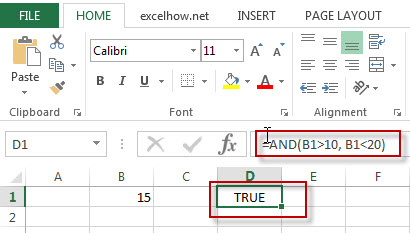### More Excel AND Function Examples

• Conditional Formatting date with red Amber or Green
How to highlight the date with red if the cell dates is past now(). How to highlight the date with amber if the cell date is past now but within the next 6 months from now(). How to highlight the date with green if the cell date is more than 6 months from now…
• Check If Multiple Cells are Equal In Excel
Assuming that you have a list of data in range A1:C1, and you want to compare if these cells are equal, if so, then return True, otherwise, return False. You need to create an Excel array formula based on the AND function and the EXACT function…